# Selina Solutions Concise Physics Class 10 Chapter 10 Electro-magnetism

Selina Solutions Concise Physics Class 10 Chapter 10 Electromagnetism explains the relationship between the magnetic field and electric field. Students often lack knowledge about the concepts covered as very often they do not understand during class hours. To get their doubts cleared instantly, students need to practise Selina Solutions on a daily basis. By referring to Selina Solutions PDF, students face the exam without fear as the answers provided here are accurate.

The expert faculty prepare  Selina Solutions Concise Physics Class 10 at BYJU’S with the aim of helping students boost their exam preparation. Selina Solutions PDF is a vital study material for students who face obstacles in solving textbook questions. These solutions help students to improve problem-solving and logical thinking abilities, required for the board exam. Download the Selina Solutions Concise Physics Class 10 PDF, links are given here for free download.

## Selina Solutions Concise Physics Chapter 10 Electromagnetism:-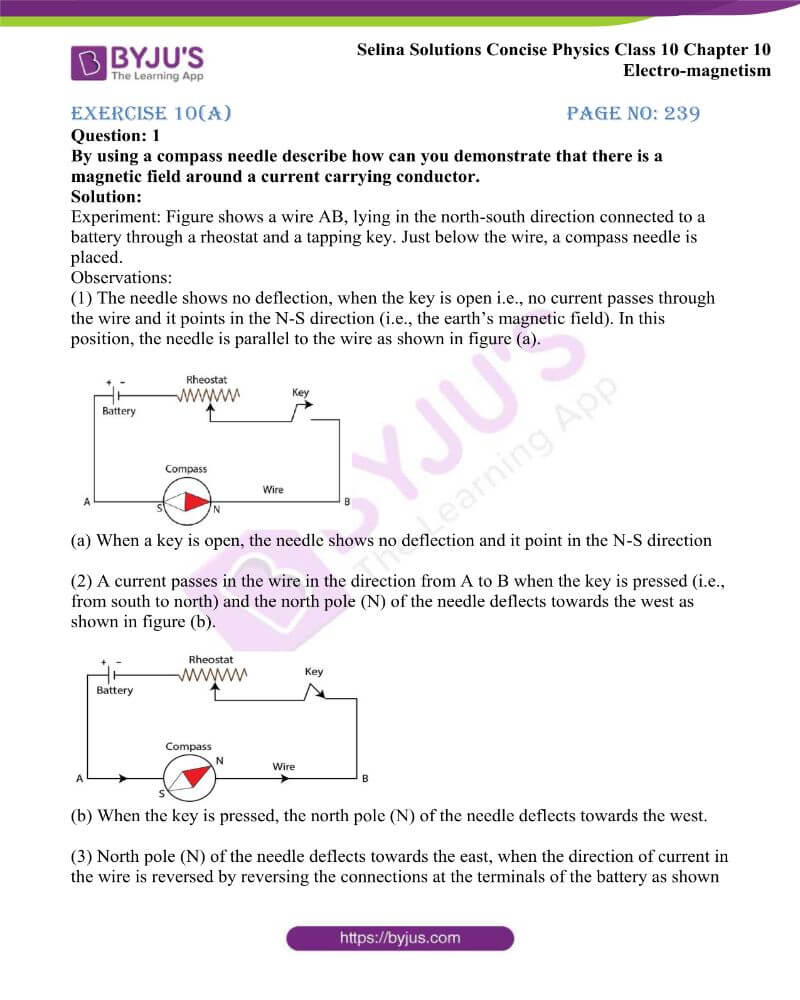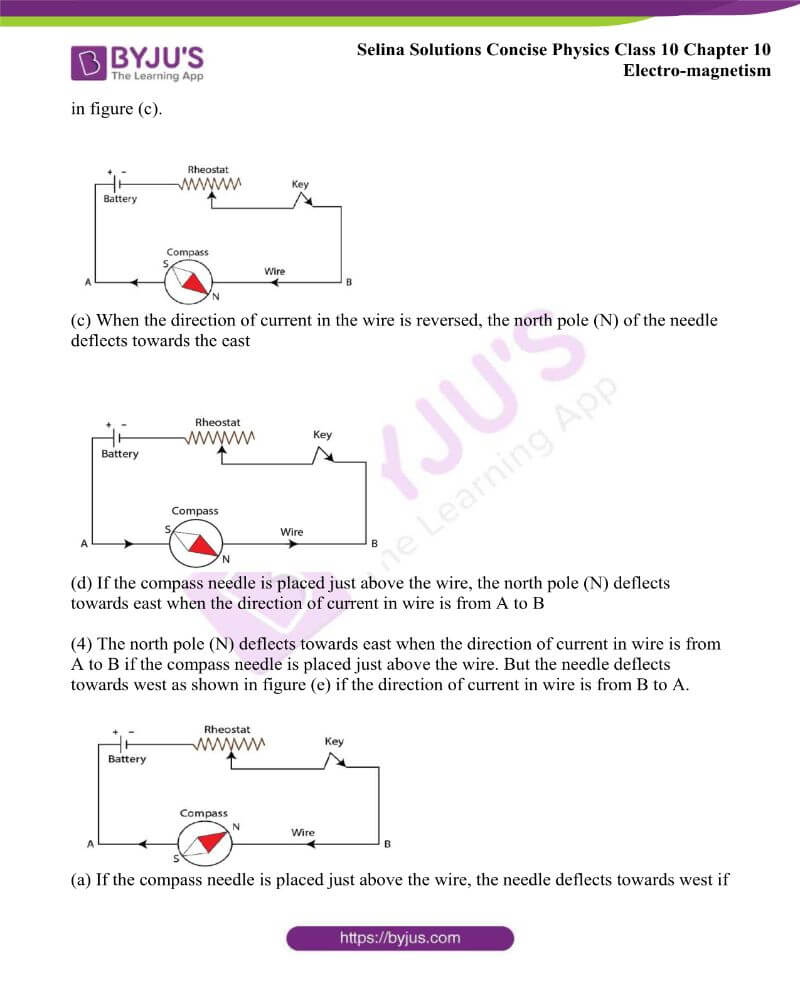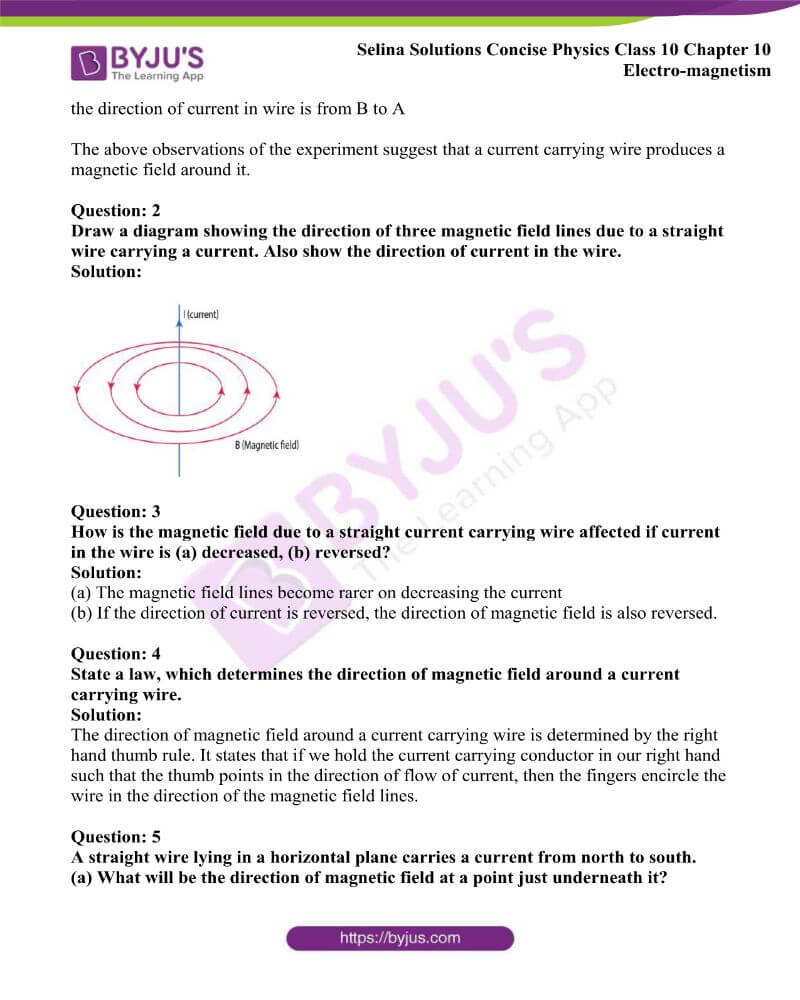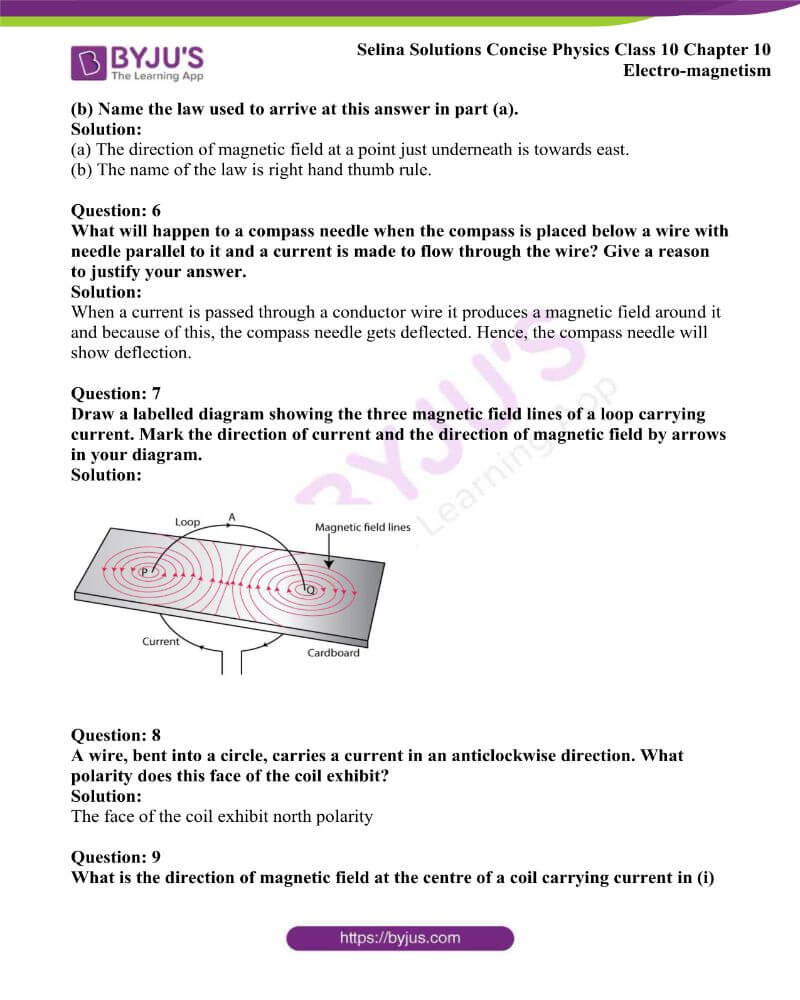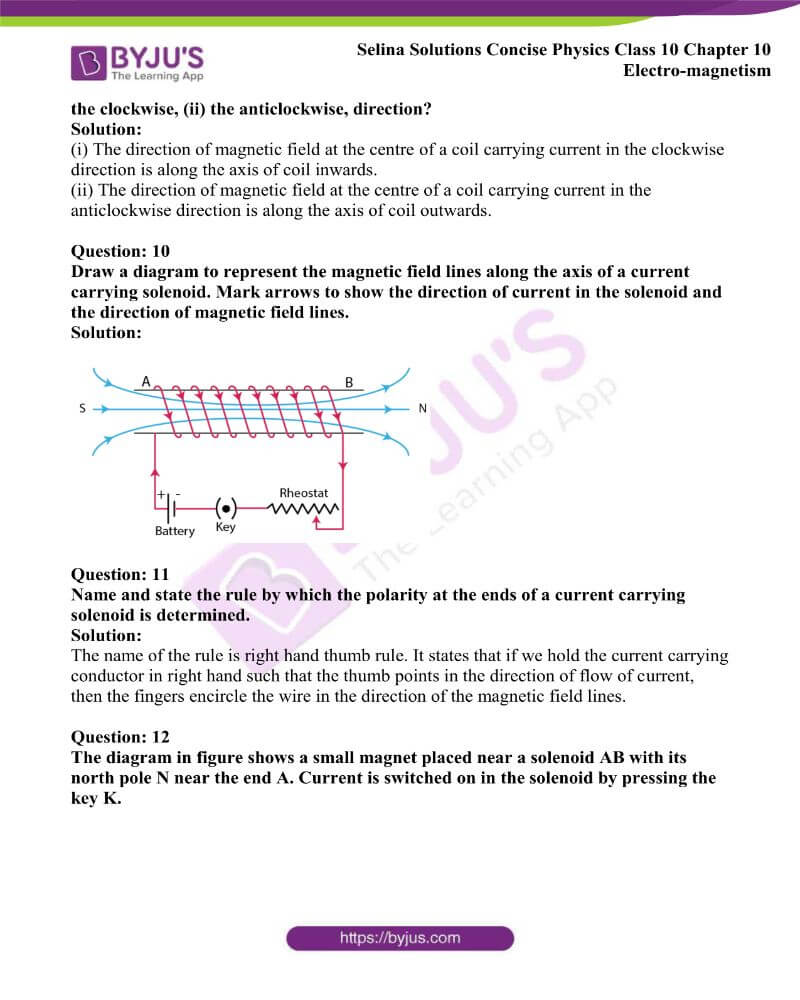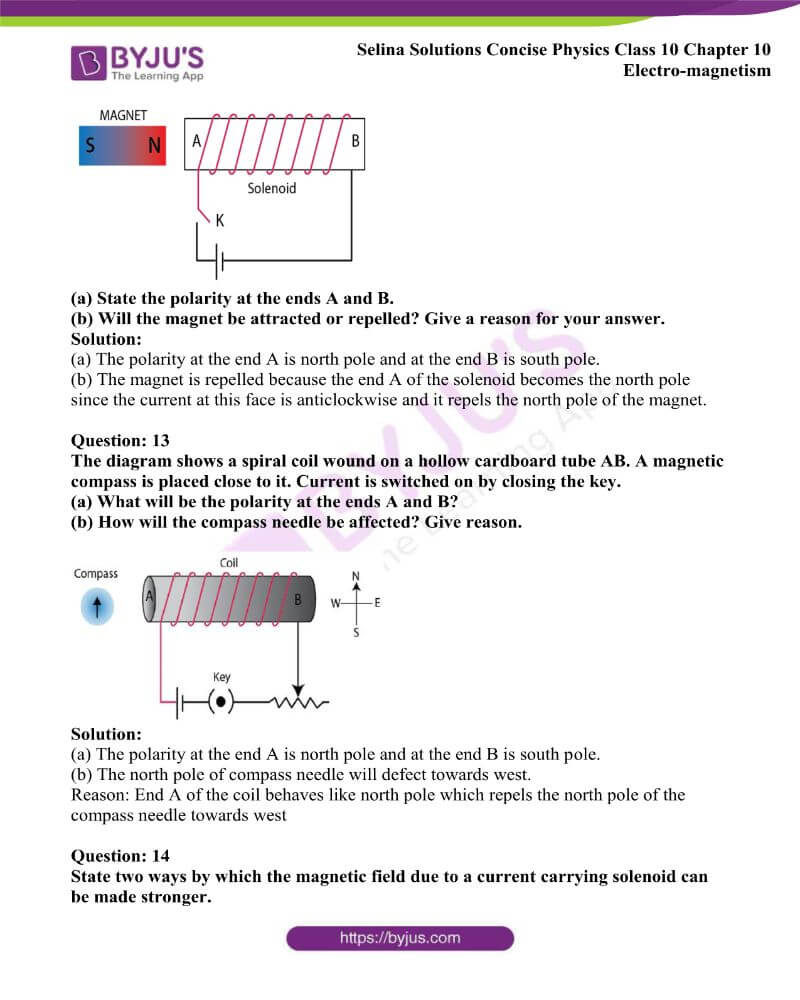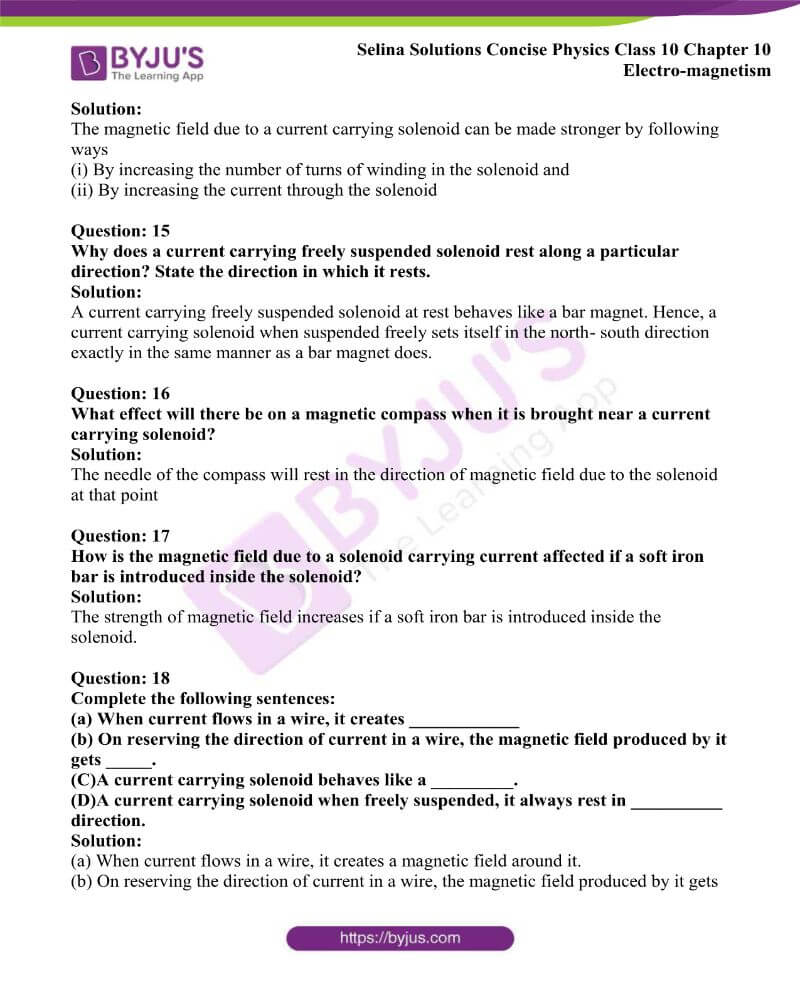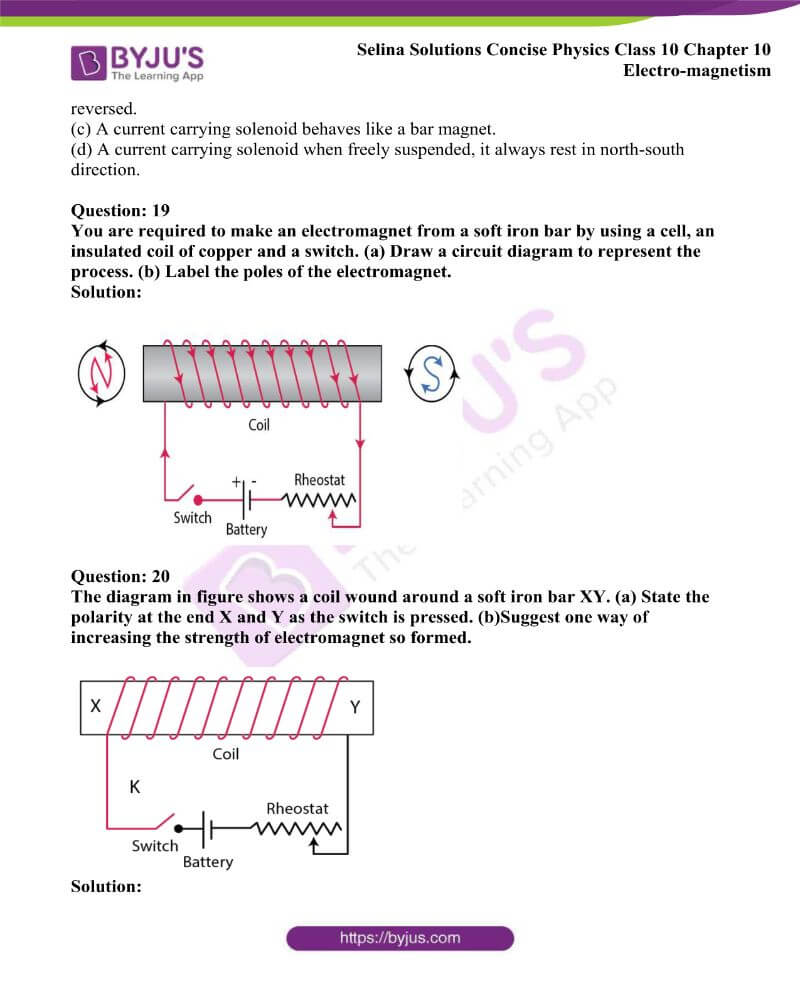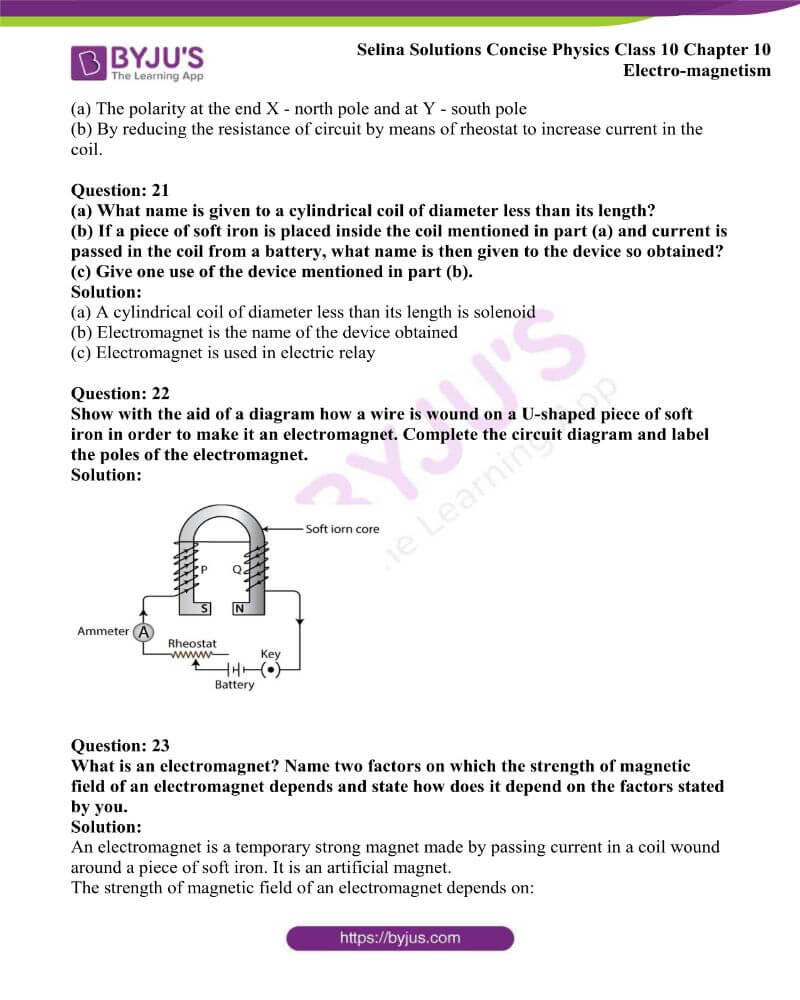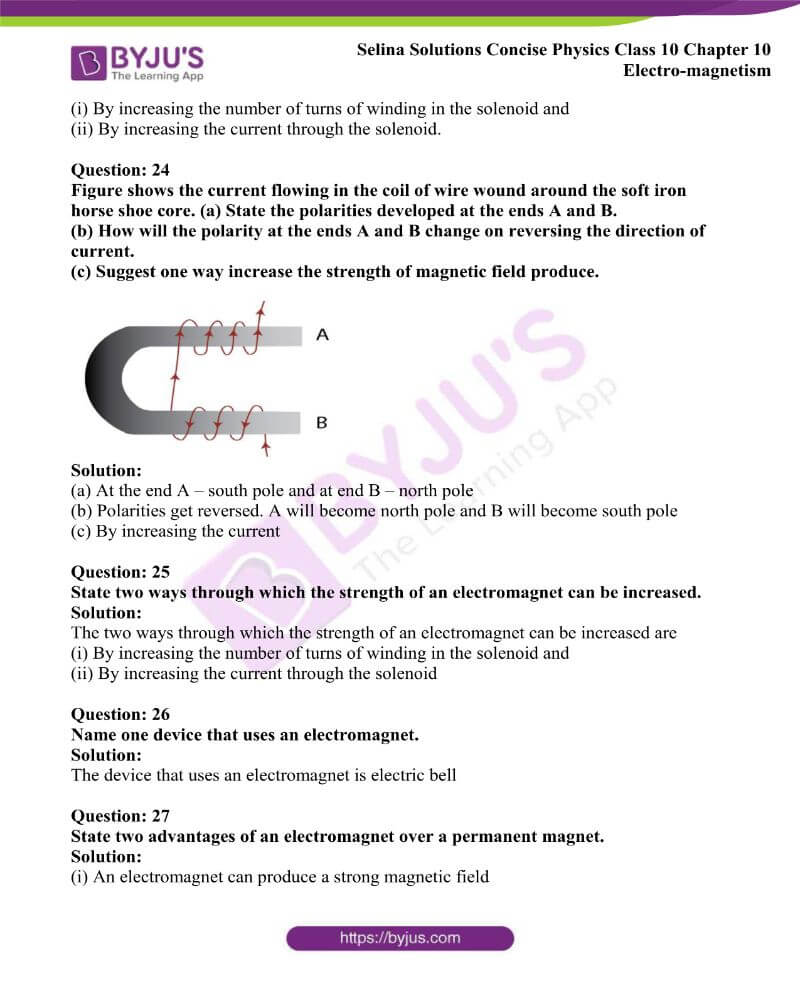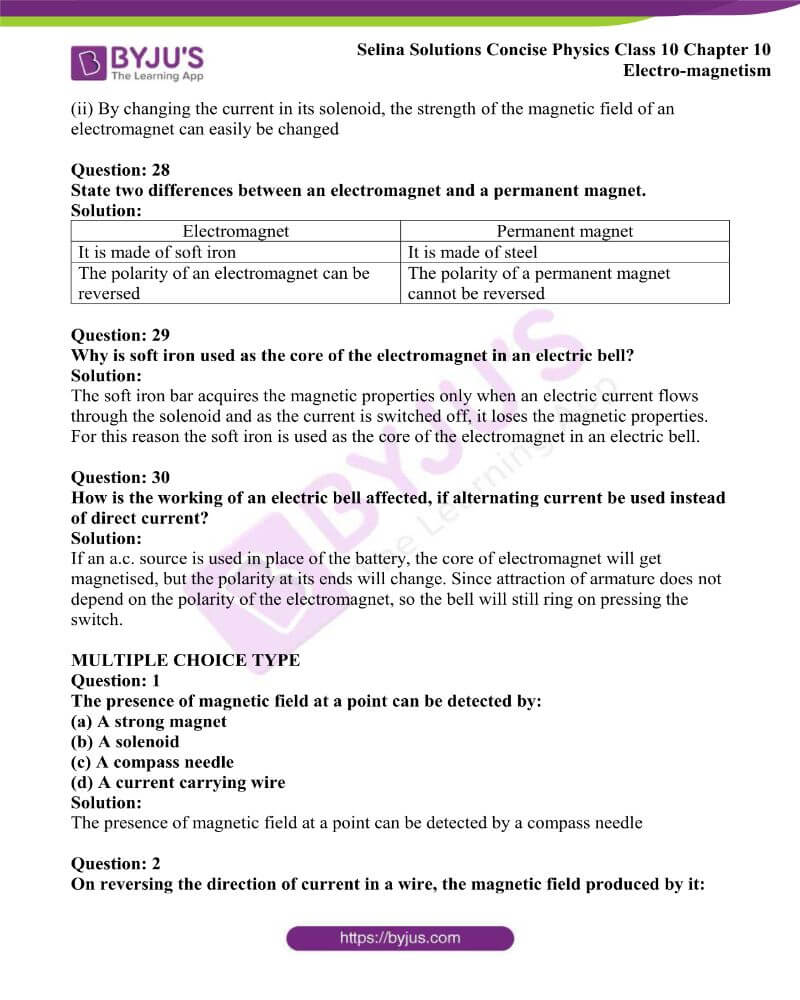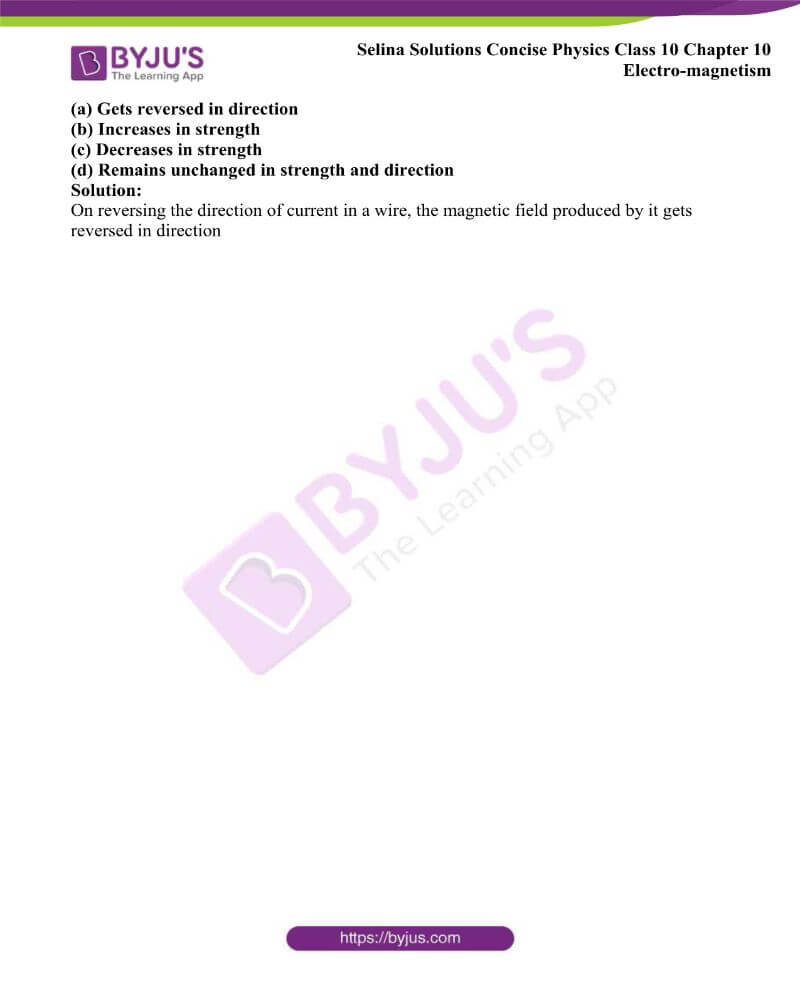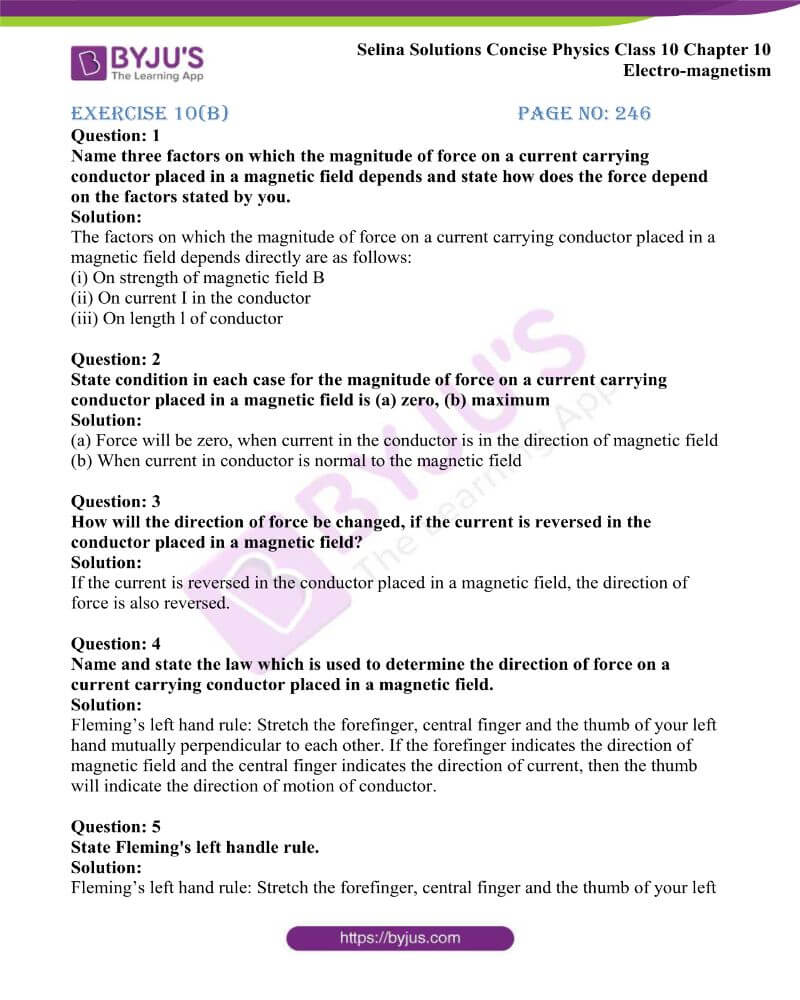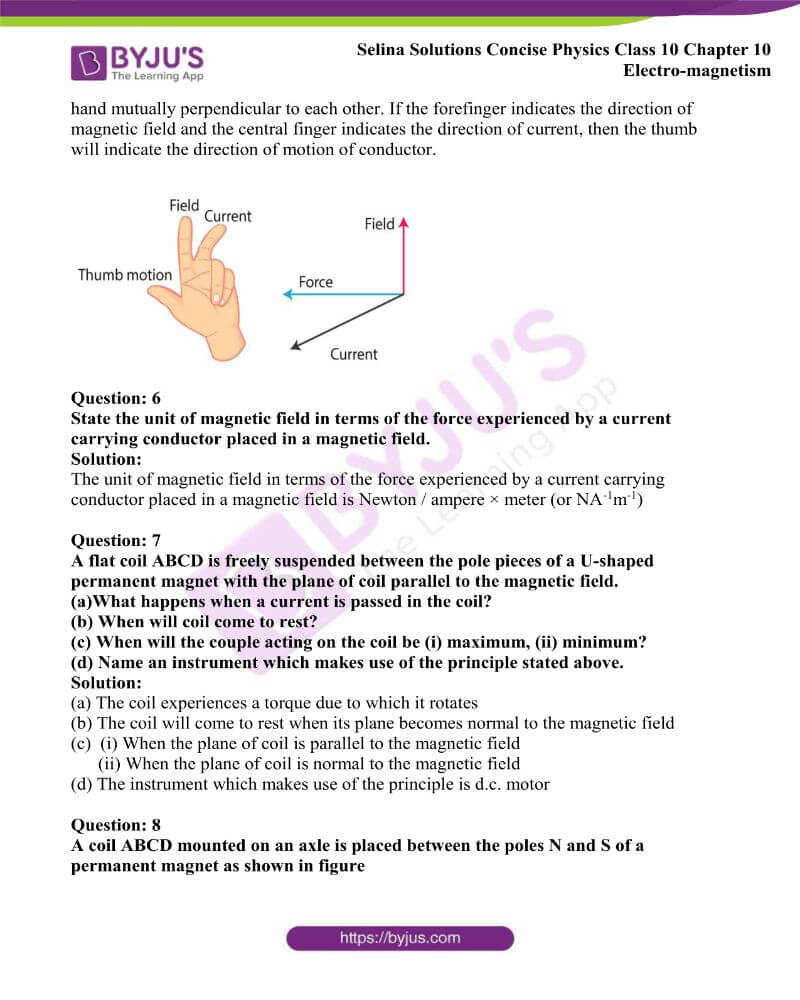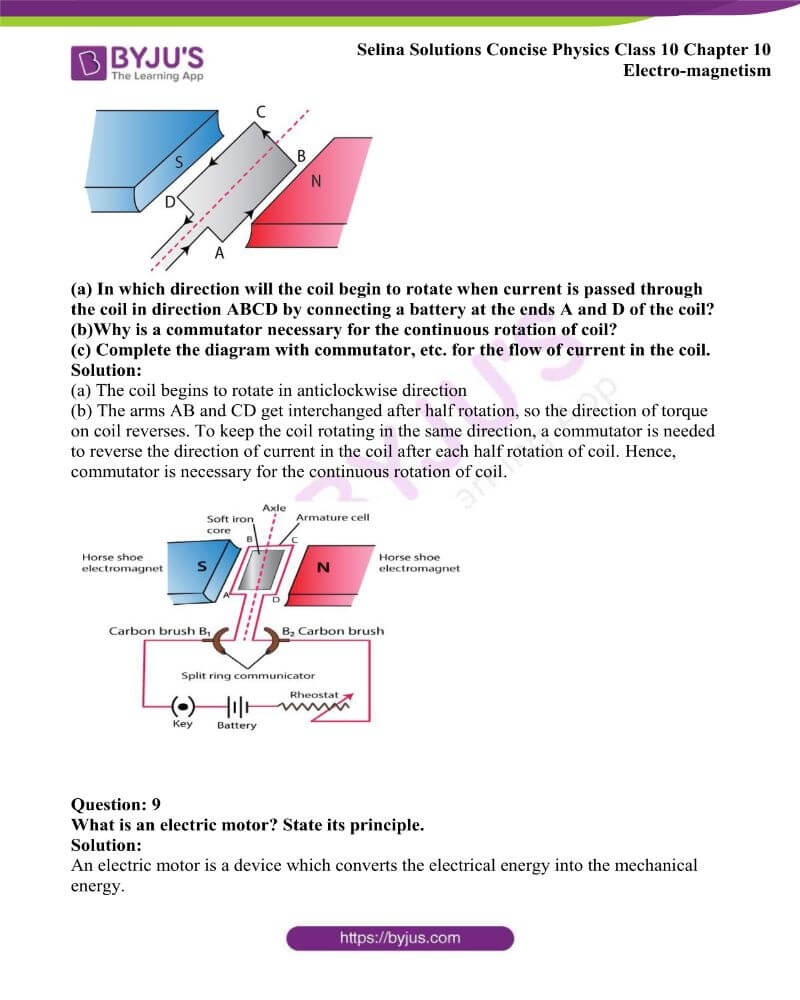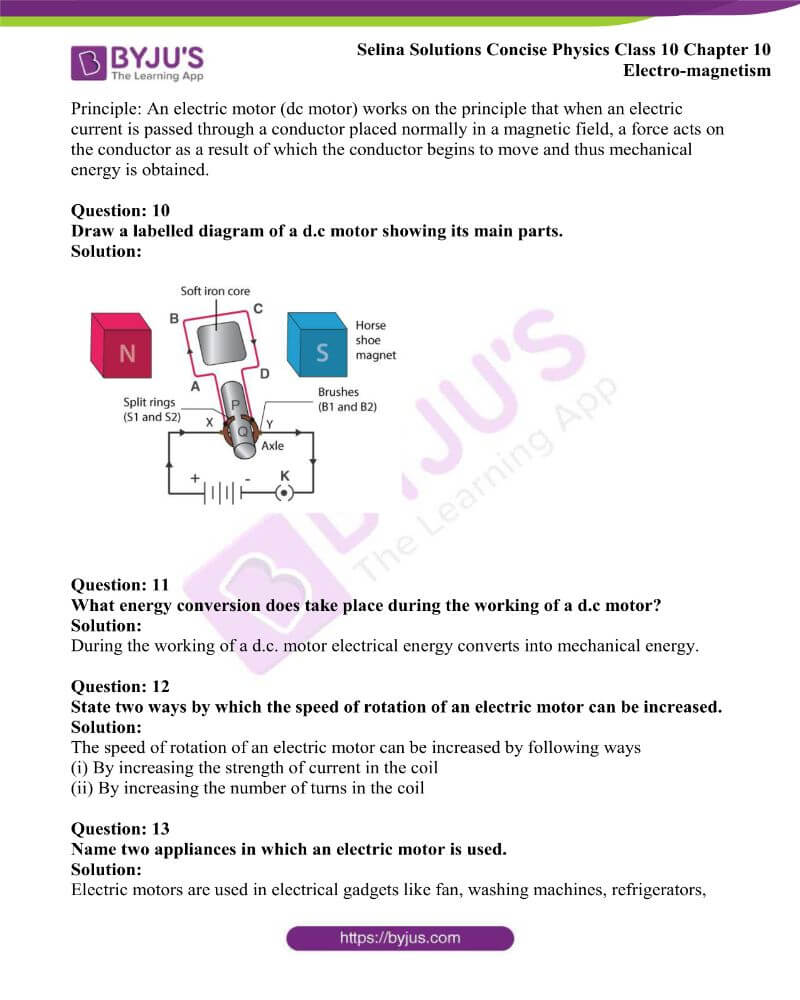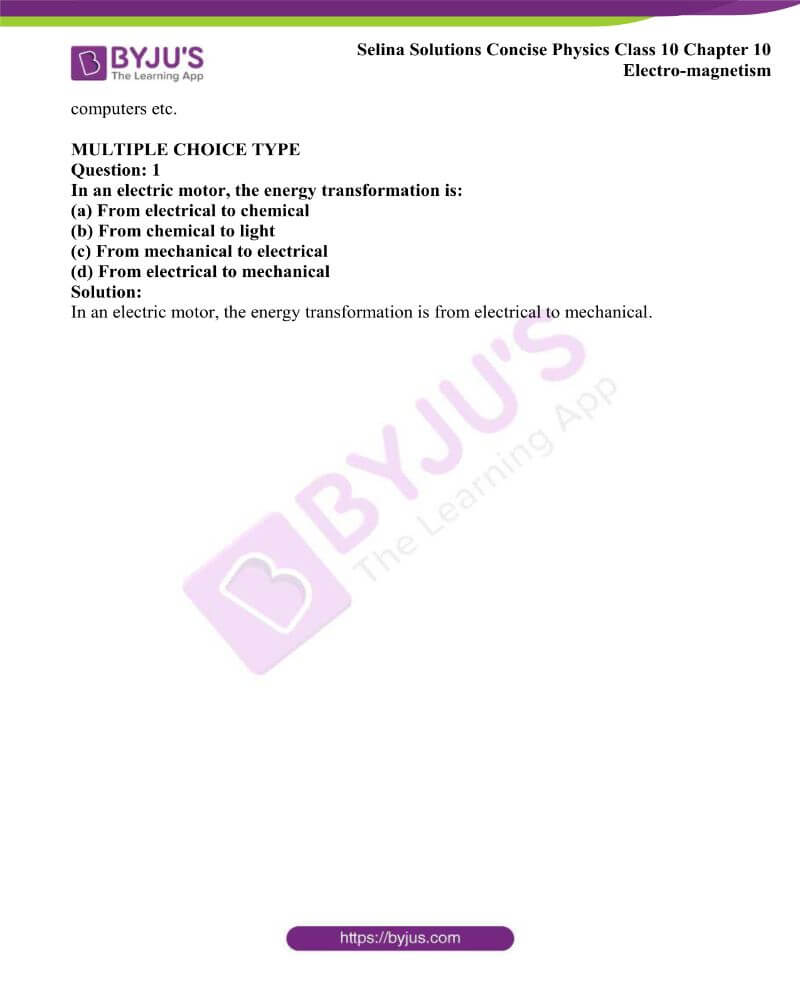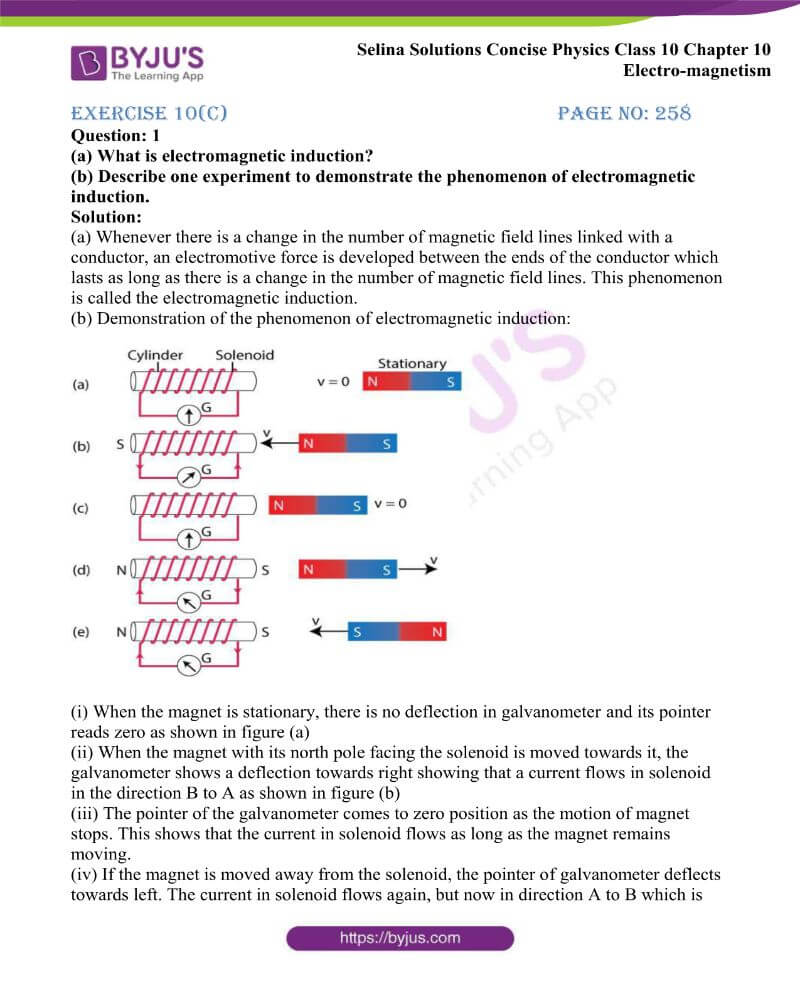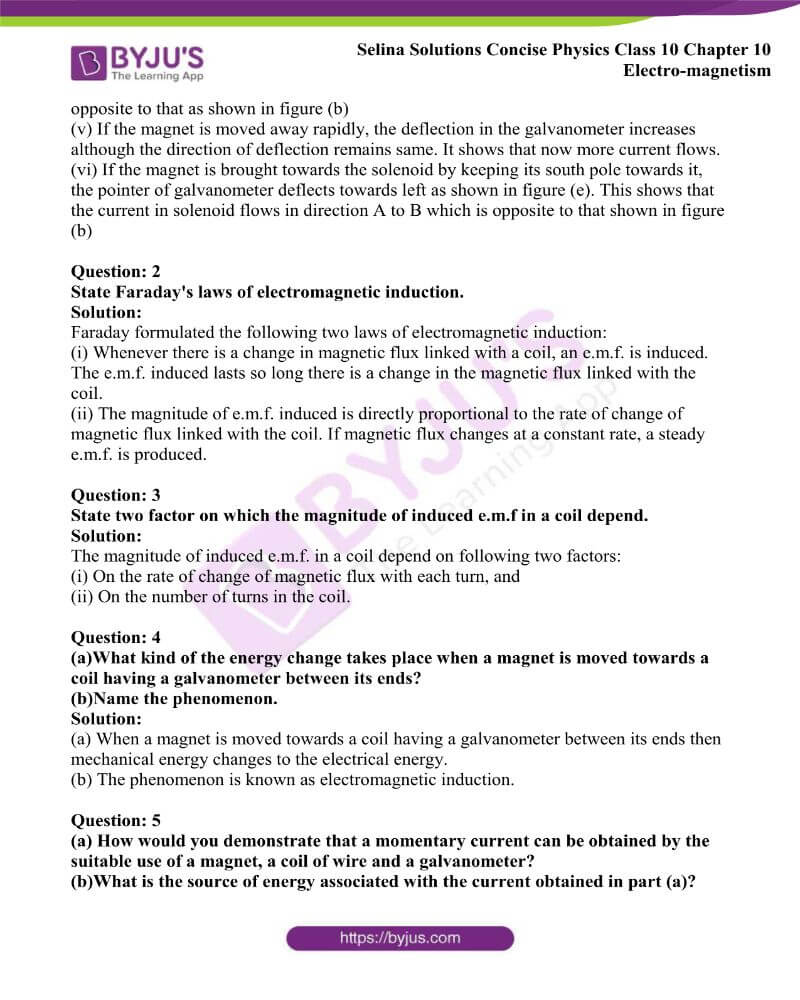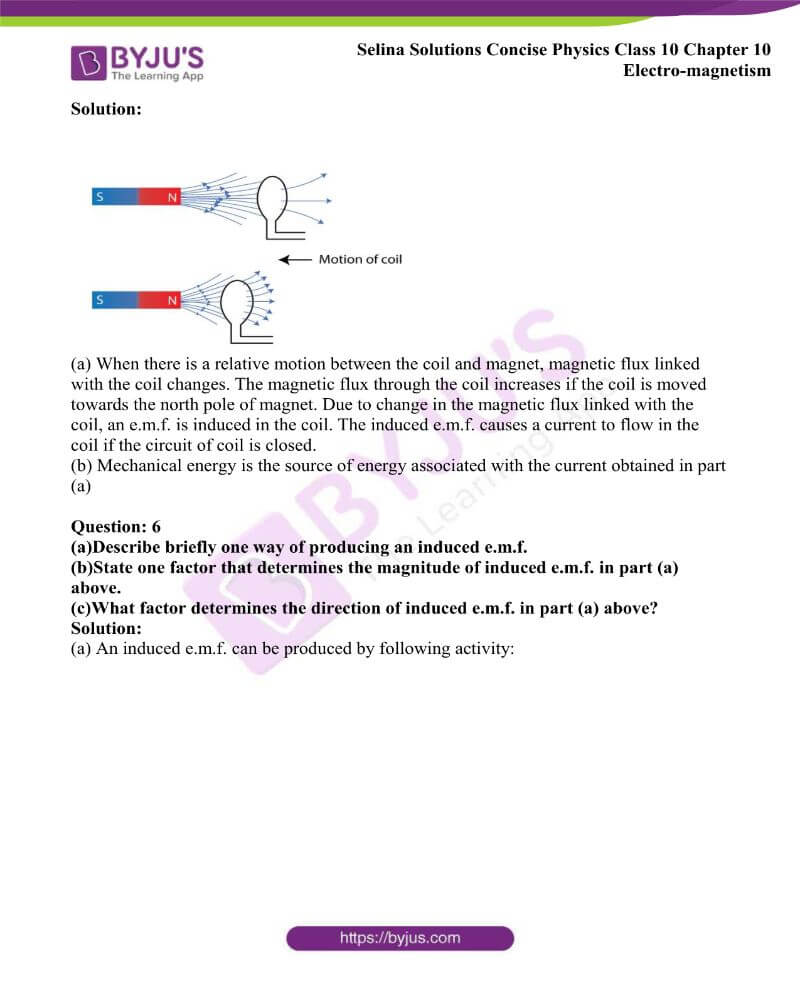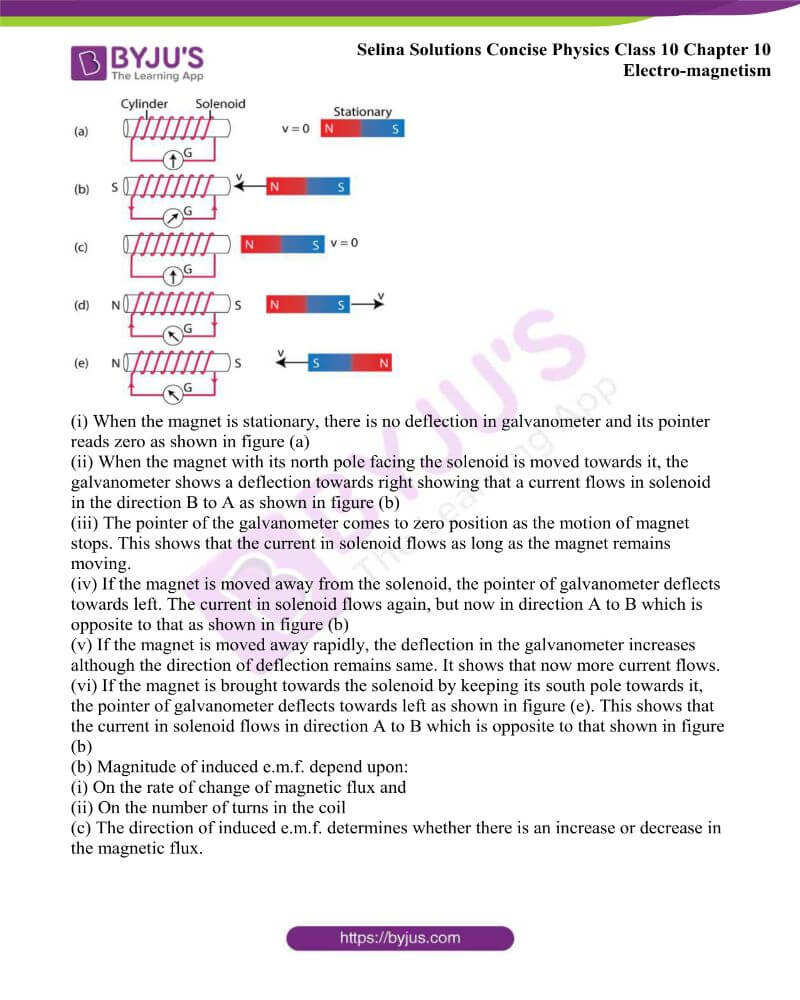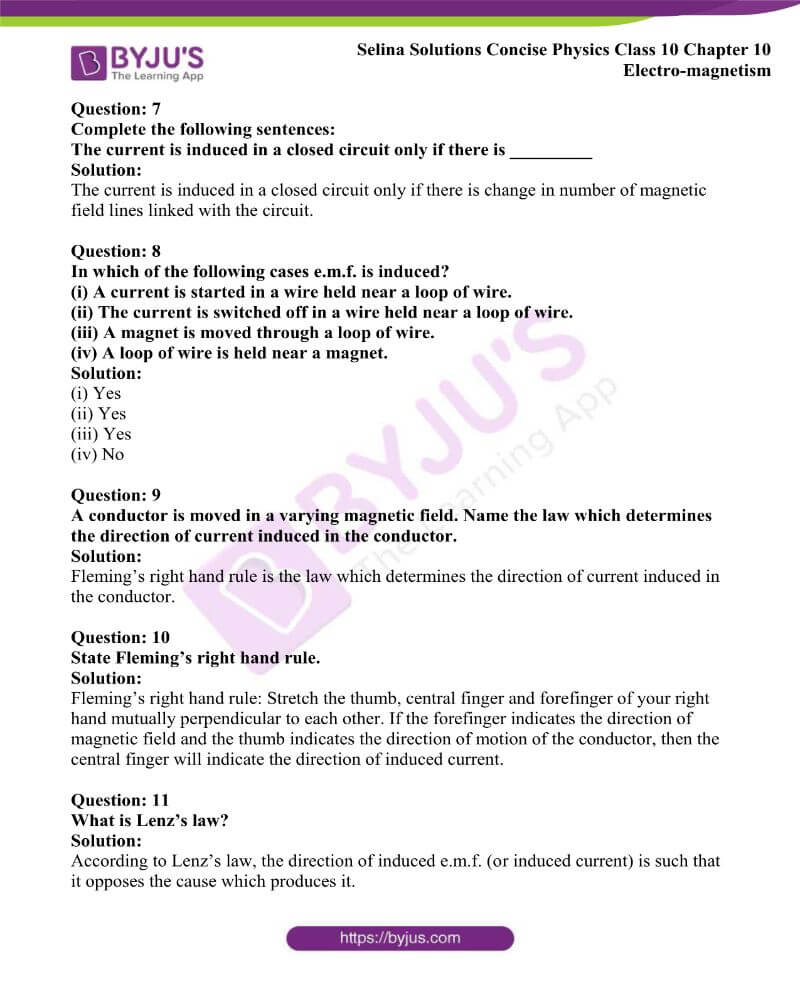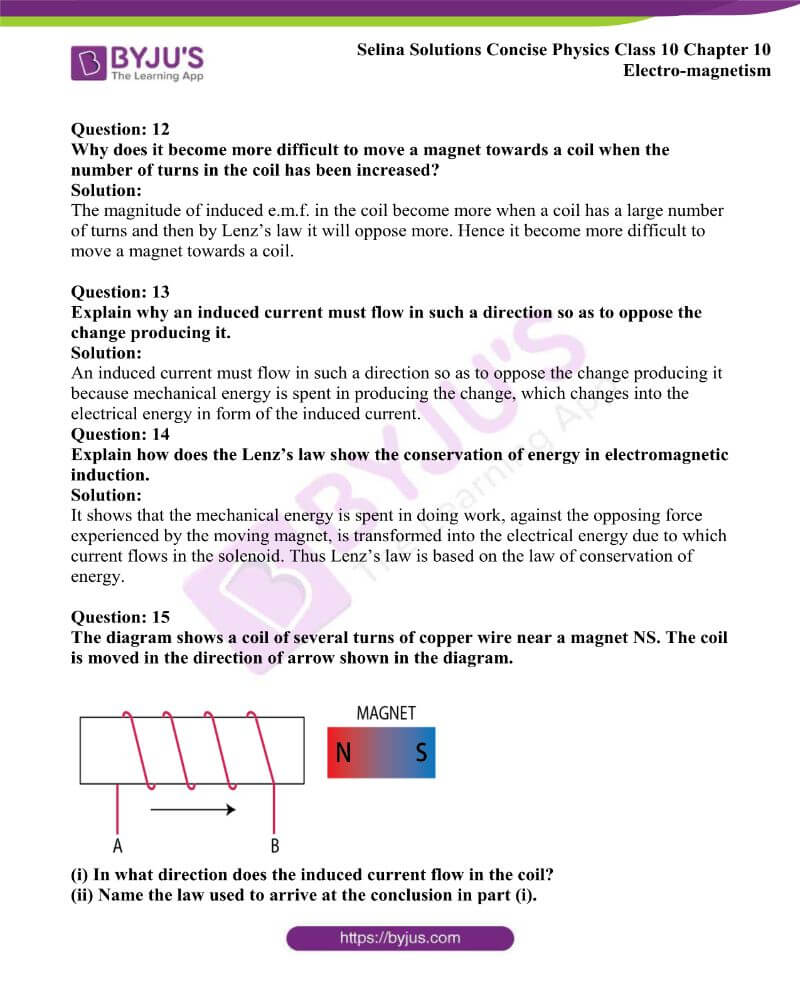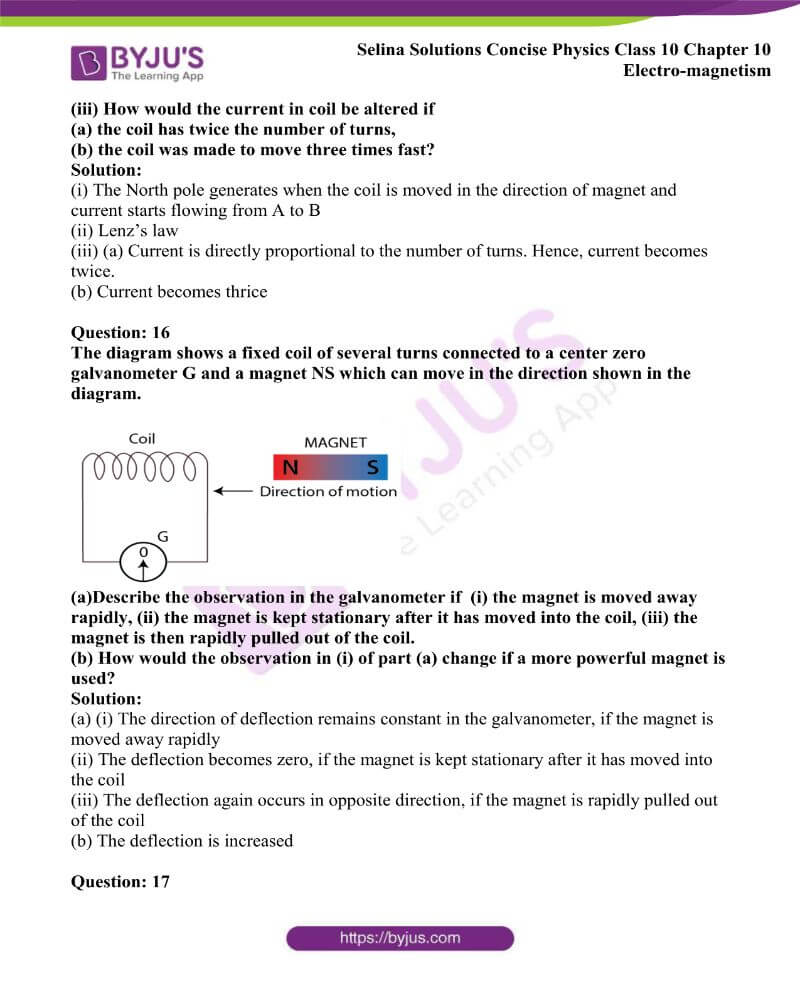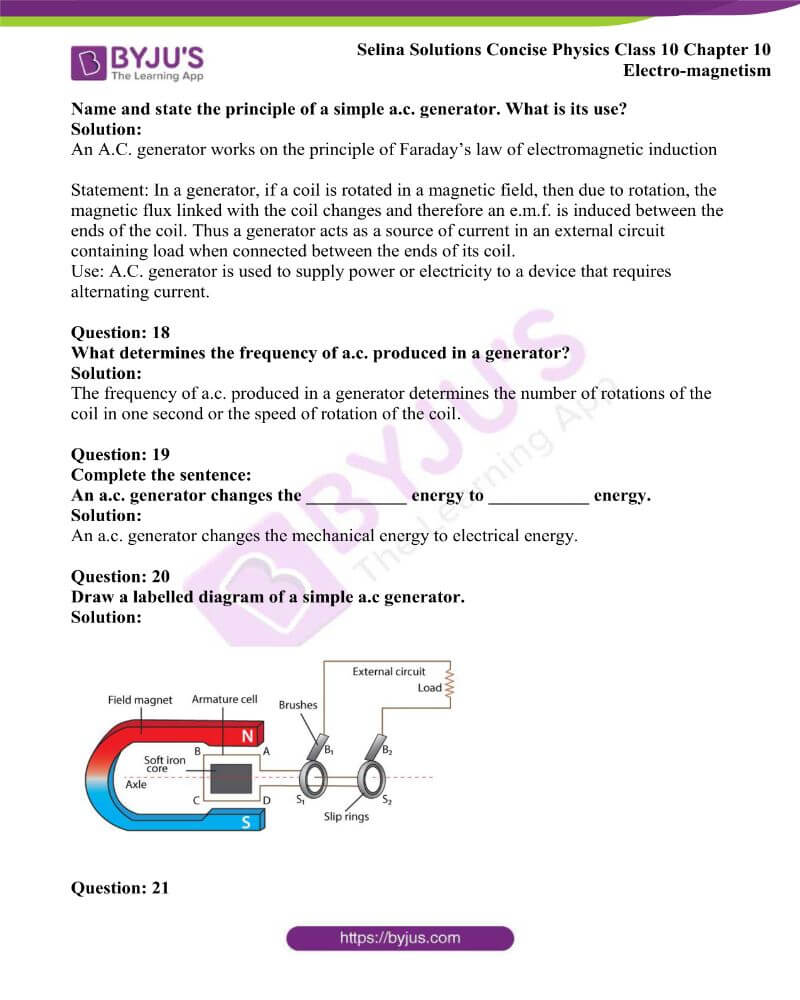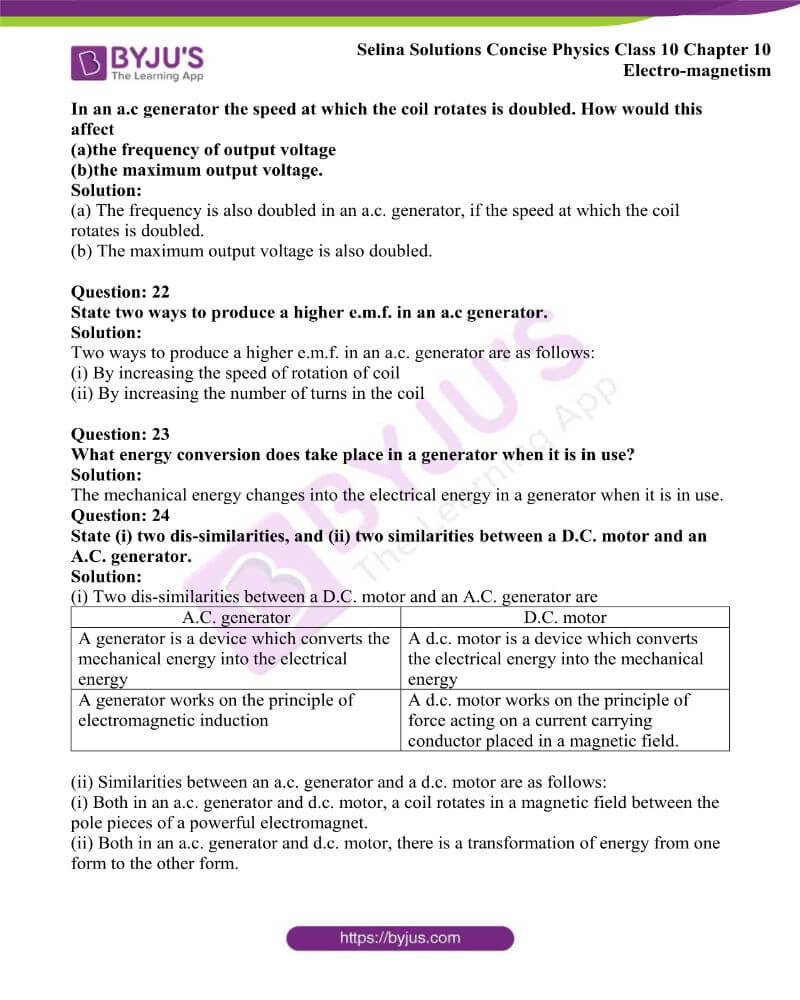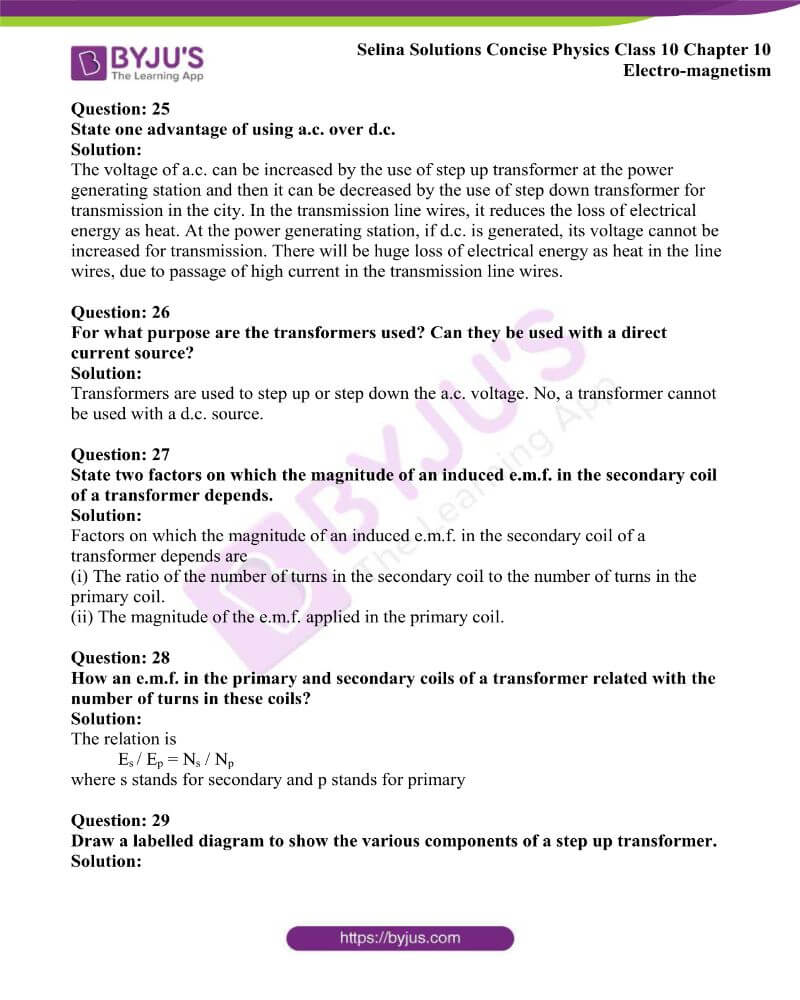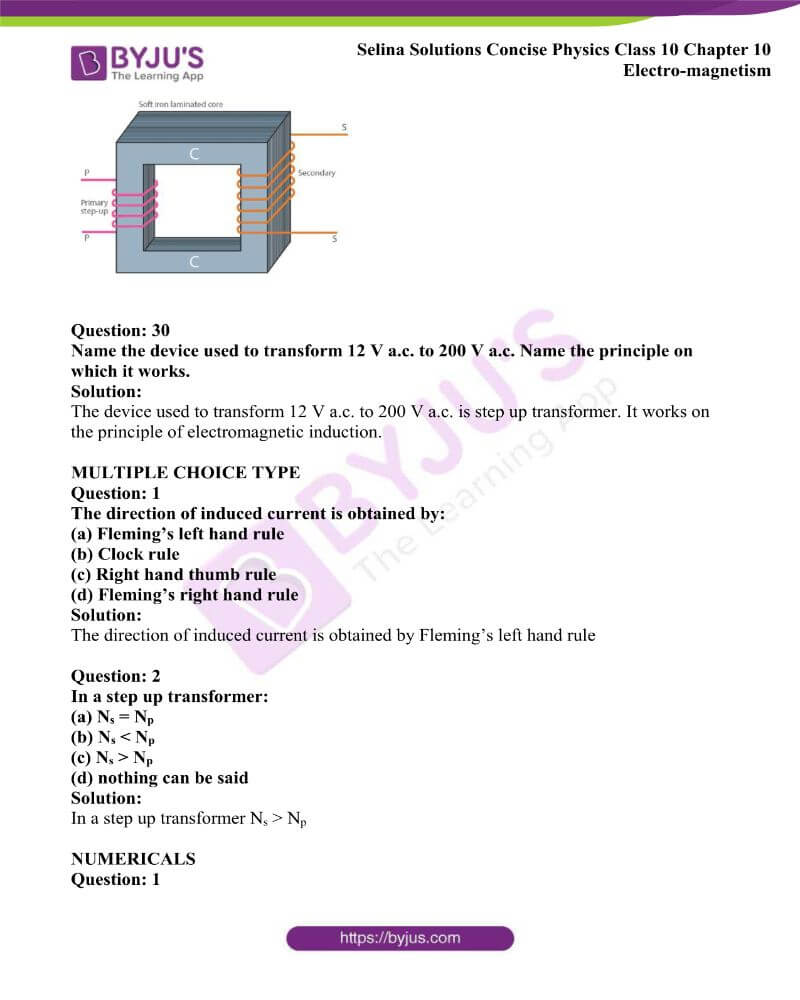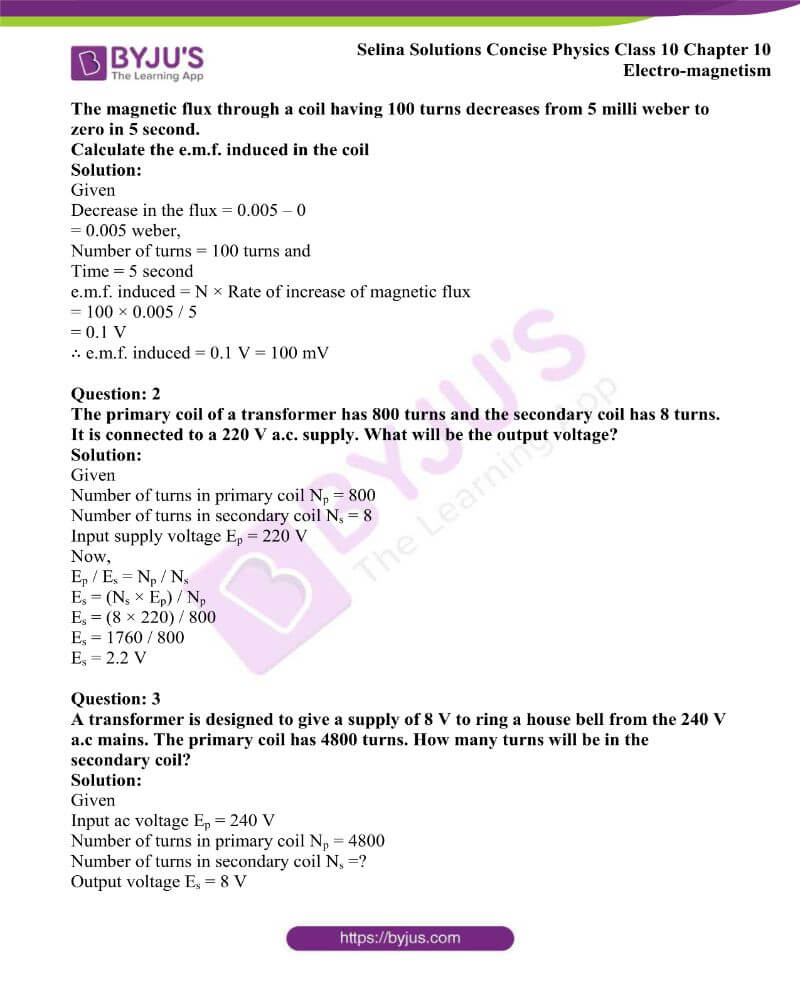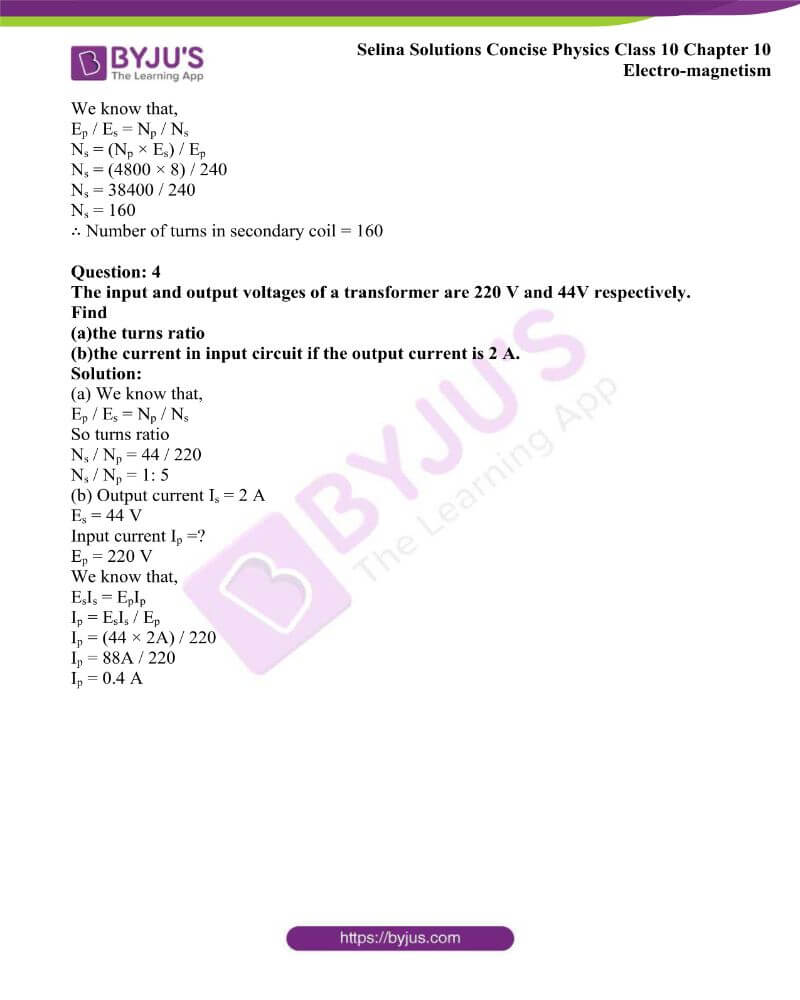### Access Answers to Physics Selina Solutions Concise Physics Class 10 Chapter 10 Electromagnetism

Exercise 10(a) page no: 239

Question: 1

By using a compass needle describe how can you demonstrate that there is a magnetic field around a current-carrying conductor.

Solution:

Experiment: Figure shows a wire AB, lying in the north-south direction connected to a battery through a rheostat and a tapping key. Just below the wire, a compass needle is placed.

Observations:

(1) The needle shows no deflection, when the key is open i.e., no current passes through the wire and it points in the N-S direction (i.e., the earth’s magnetic field). In this position, the needle is parallel to the wire as shown in figure (a).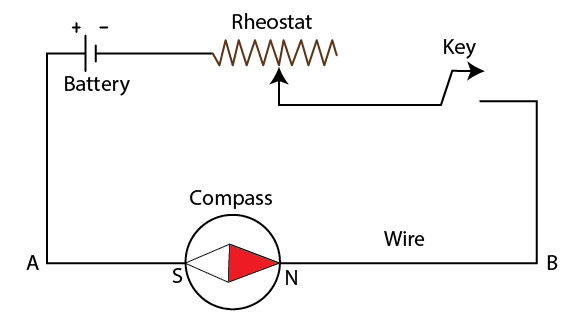(a) When a key is open, the needle shows no deflection and it point in the N-S direction

(2) A current passes in the wire in the direction from A to B when the key is pressed (i.e., from south to north) and the north pole (N) of the needle deflects towards the west as shown in figure (b).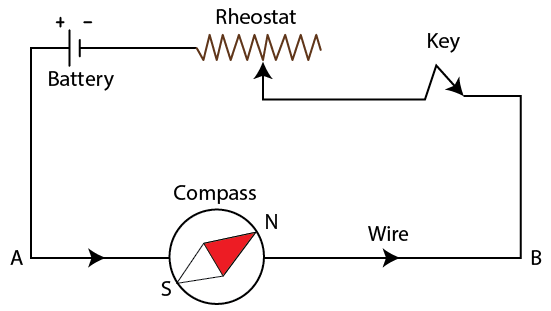(b) When the key is pressed, the north pole (N) of the needle deflects towards the west.

(3) North pole (N) of the needle deflects towards the east, when the direction of current in the wire is reversed by reversing the connections at the terminals of the battery as shown in figure (c).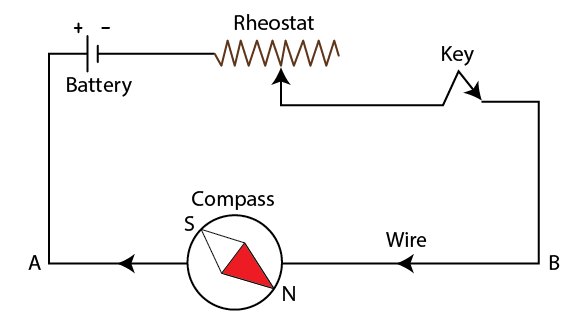(c) When the direction of current in the wire is reversed, the north pole (N) of the needle deflects towards the east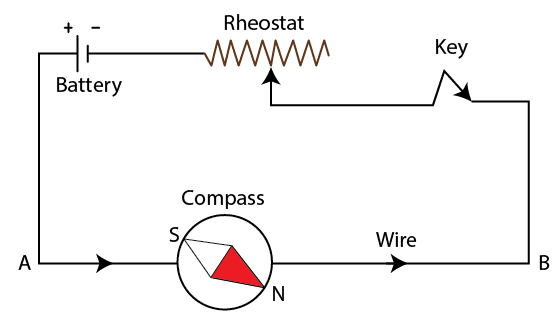(d) If the compass needle is placed just above the wire, the north pole (N) deflects towards the east when the direction of current in the wire is from A to B

(4) The north pole (N) deflects towards the east when the direction of current in the wire is from A to B if the compass needle is placed just above the wire. But the needle deflects towards the west as shown in figure (e) if the direction of current in the wire is from B to A.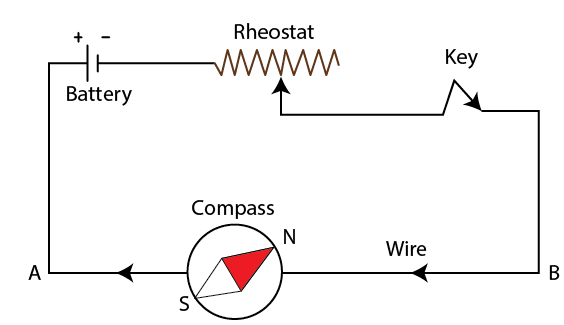(a) If the compass needle is placed just above the wire, the needle deflects towards the west if the direction of current in the wire is from B to A

The above observations of the experiment suggest that a current-carrying wire produces a magnetic field around it.

Question: 2

Draw a diagram showing the direction of three magnetic field lines due to a straight wire carrying a current. Also show the direction of current in the wire.

Solution: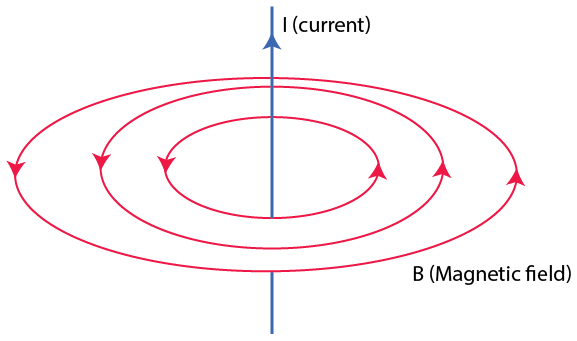Question: 3

How is the magnetic field due to a straight current-carrying wire affected if current in the wire is (a) decreased, (b) reversed?

Solution:

(a) The magnetic field lines become rarer on decreasing the current

(b) If the direction of current is reversed, the direction of the magnetic field is also reversed.

Question: 4

State a law, which determines the direction of the magnetic field around a current-carrying wire.

Solution:

The direction of the magnetic field around a current-carrying wire is determined by the right-hand thumb rule. It states that if we hold the current-carrying conductor in our right hand such that the thumb points in the direction of flow of current, then the fingers encircle the wire in the direction of the magnetic field lines.

Question: 5

A straight wire lying in a horizontal plane carries a current from north to south.

(a) What will be the direction of the magnetic field at a point just underneath it?

(b) Name the law used to arrive at this answer in part (a).

Solution:

(a) The direction of the magnetic field at a point just underneath is towards east.

(b) The name of the law is the right-hand thumb rule.

Question: 6

What will happen to a compass needle when the compass is placed below a wire with needle parallel to it and a current is made to flow through the wire? Give a reason to justify your answer.

Solution:

When a current is passed through a conductor wire it produces a magnetic field around it and because of this, the compass needle gets deflected. Hence, the compass needle will show deflection.

Question: 7

Draw a labelled diagram showing the three magnetic field lines of a loop carrying current. Mark the direction of current and the direction of the magnetic field by arrows in your diagram.

Solution: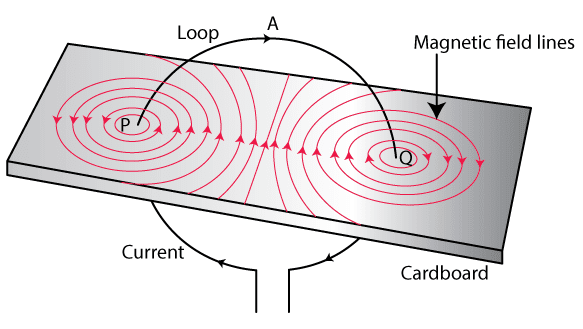Question: 8

A wire, bent into a circle, carries a current in an anticlockwise direction. What polarity does this face of the coil exhibit?

Solution:

The face of the coil exhibit north polarity

Question: 9

What is the direction of the magnetic field at the centre of a coil carrying current in (i) the clockwise, (ii) the anticlockwise, direction?

Solution:

(i) The direction of the magnetic field at the centre of a coil carrying current in the clockwise direction is along the axis of coil inwards.

(ii) The direction of the magnetic field at the centre of a coil carrying current in the anticlockwise direction is along the axis of coil outwards.

Question: 10

Draw a diagram to represent the magnetic field lines along the axis of a current-carrying solenoid. Mark arrows to show the direction of current in the solenoid and the direction of magnetic field lines.

Solution: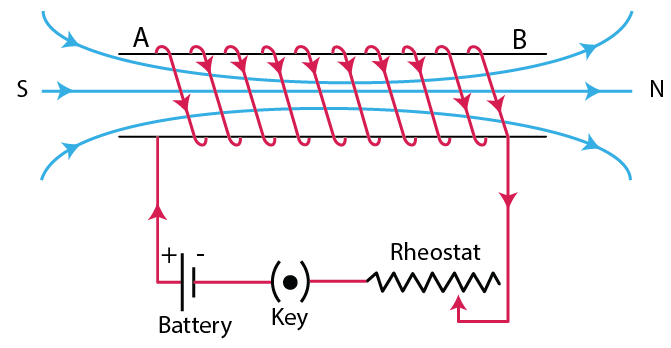Question: 11

Name and state the rule by which the polarity at the ends of a current carrying solenoid is determined.

Solution:

The name of the rule is the right hand thumb rule. It states that if we hold the current-carrying conductor in right hand such that the thumb points in the direction of flow of current, then the fingers encircle the wire in the direction of the magnetic field lines.

Question: 12

The diagram in the figure shows a small magnet placed near a solenoid AB with its north pole N near the end A. Current is switched on in the solenoid by pressing the key K.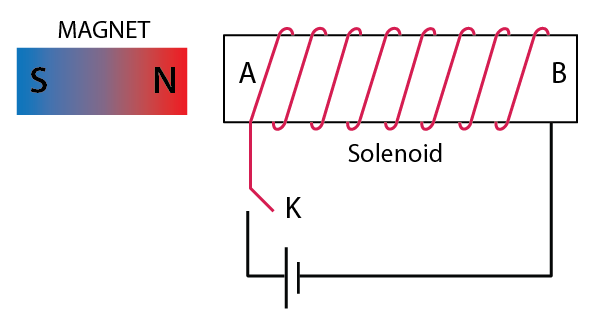(a) State the polarity at the ends A and B.

(b) Will the magnet be attracted or repelled? Give a reason for your answer.

Solution:

(a) The polarity at the end A is north pole and at the end B is the south pole.

(b) The magnet is repelled because the end A of the solenoid becomes the north pole since the current at this face is anticlockwise and it repels the north pole of the magnet.

Question: 13

The diagram shows a spiral coil wound on a hollow cardboard tube AB. A magnetic compass is placed close to it. Current is switched on by closing the key.

(a) What will be the polarity at the ends A and B?

(b) How will the compass needle be affected? Give reason.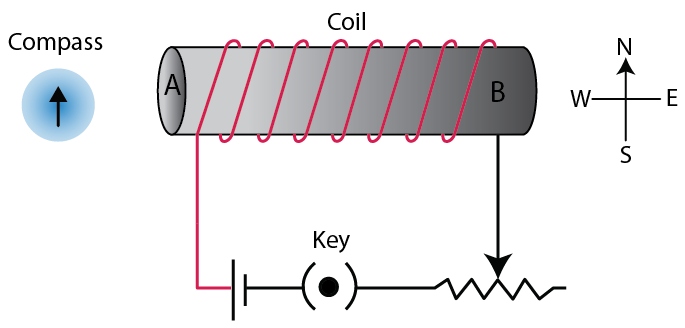Solution:

(a) The polarity at the end A is the north pole and at the end, B is the south pole.

(b) The north pole of the compass needle will defect towards west.

Reason: End A of the coil behaves like north pole which repels the north pole of the compass needle towards the west

Question: 14

State two ways by which the magnetic field due to a current-carrying solenoid can be made stronger.

Solution:

The magnetic field due to a current-carrying solenoid can be made stronger by following ways

(i) By increasing the number of turns of winding in the solenoid and

(ii) By increasing the current through the solenoid

Question: 15

Why does a current-carrying freely suspended solenoid rest along a particular direction? State the direction in which it rests.

Solution:

A current-carrying freely suspended solenoid at rest behaves like a bar magnet. Hence, a current-carrying solenoid when suspended freely sets itself in the north-south direction exactly in the same manner as a bar magnet does.

Question: 16

What effect will there be on a magnetic compass when it is brought near a current-carrying solenoid?

Solution:

The needle of the compass will rest in the direction of the magnetic field due to the solenoid at that point

Question: 17

How is the magnetic field due to a solenoid carrying current affected if a soft iron bar is introduced inside the solenoid?

Solution:

The strength of the magnetic field increases if a soft iron bar is introduced inside the solenoid.

Question: 18

Complete the following sentences:

(a) When current flows in a wire, it creates ____________

(b) On reserving the direction of current in a wire, the magnetic field produced by it gets _____.

(C)A current carrying solenoid behaves like a _________.

(D)A current-carrying solenoid when freely suspended, it always rest in __________ direction.

Solution:

(a) When current flows in a wire, it creates a magnetic field around it.

(b) On reserving the direction of current in a wire, the magnetic field produced by it gets reversed.

(c) A current-carrying solenoid behaves like a bar magnet.

(d) A current-carrying solenoid when freely suspended, it always rests in a north-south direction.

Question: 19

You are required to make an electromagnet from a soft iron bar by using a cell, an insulated coil of copper and a switch. (a) Draw a circuit diagram to represent the process. (b) Label the poles of the electromagnet.

Solution: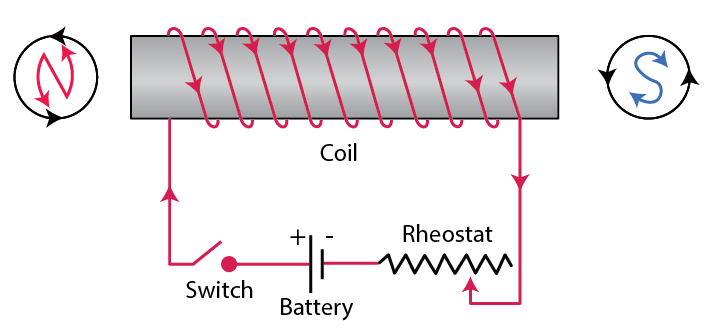Question: 20

The diagram in the figure shows a coil wound around a soft iron bar XY. (a) State the polarity at the end X and Y as the switch is pressed. (b)Suggest one way of increasing the strength of electromagnet so formed.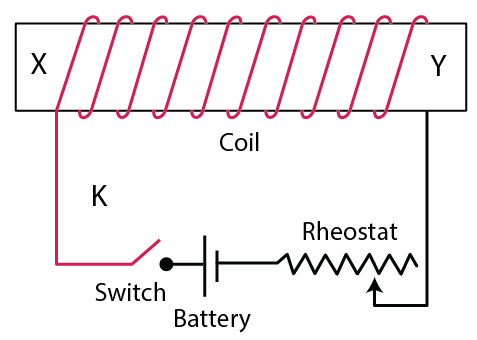Solution:

(a) The polarity at the end X – north pole and at Y – south pole

(b) By reducing the resistance of the circuit by means of the rheostat to increase the current in the coil.

Question: 21

(a) What name is given to a cylindrical coil of diameter less than its length?

(b) If a piece of soft iron is placed inside the coil mentioned in part (a) and current is passed in the coil from a battery, what name is then given to the device so obtained?

(c) Give one use of the device mentioned in part (b).

Solution:

(a) A cylindrical coil of diameter less than its length is solenoid.

(b) Electromagnet is the name of the device obtained.

(c) Electromagnet is used in electric relay.

Question: 22

Show with the aid of a diagram how a wire is wound on a U-shaped piece of soft iron in order to make it an electromagnet. Complete the circuit diagram and label the poles of the electromagnet.

Solution: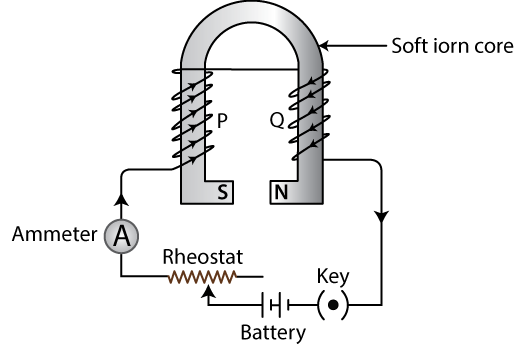Question: 23

What is an electromagnet? Name two factors on which the strength of the magnetic field of an electromagnet depends and state how does it depend on the factors stated by you.

Solution:

An electromagnet is a temporary strong magnet made by passing current in a coil wound around a piece of soft iron. It is an artificial magnet.

The strength of the magnetic field of an electromagnet depends on:

(i) By increasing the number of turns of winding in the solenoid and

(ii) By increasing the current through the solenoid.

Question: 24

Figure shows the current flowing in the coil of wire wound around the soft iron horseshoe core. (a) State the polarities developed at the ends A and B.

(b) How will the polarity at the ends A and B change on reversing the direction of current?
(c) Suggest one-way increase the strength of magnetic field produce.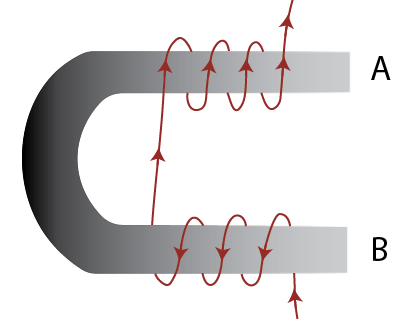Solution:

(a) At the end A – south pole and at end B – north pole

(b) Polarities get reversed. A will become north pole and B will become south pole

(c) By increasing the current

Question: 25

State two ways through which the strength of an electromagnet can be increased.

Solution:

The two ways through which the strength of an electromagnet can be increased are

(i) By increasing the number of turns of winding in the solenoid and

(ii) By increasing the current through the solenoid

Question: 26

Name one device that uses an electromagnet.

Solution:

The device that uses an electromagnet is electric bell

Question: 27

State two advantages of an electromagnet over a permanent magnet.

Solution:

(i) An electromagnet can produce a strong magnetic field

(ii) By changing the current in its solenoid, the strength of the magnetic field of an electromagnet can easily be changed

Question: 28

State two differences between an electromagnet and a permanent magnet.

Solution:

 Electromagnet Permanent magnet It is made of soft iron It is made of steel The polarity of an electromagnet can be reversed The polarity of a permanent magnet cannot be reversed

Question: 29

Why is soft iron used as the core of the electromagnet in an electric bell?

Solution:

The soft iron bar acquires the magnetic properties only when an electric current flows through the solenoid and as the current is switched off, it loses the magnetic properties. For this reason, the soft iron is used as the core of the electromagnet in an electric bell.

Question: 30

How is the working of an electric bell affected, if alternating current be used instead of direct current?

Solution:

If an a.c. source is used in place of the battery, the core of electromagnet will get magnetised, but the polarity at its ends will change. Since the attraction of armature does not depend on the polarity of the electromagnet, so the bell will still ring on pressing the switch.

MULTIPLE CHOICE TYPE

Question: 1

The presence of the magnetic field at a point can be detected by:

(a) A strong magnet

(b) A solenoid

(c) A compass needle

(d) A current-carrying wire

Solution:

The presence of a magnetic field at a point can be detected by a compass needle

Question: 2

On reversing the direction of current in a wire, the magnetic field produced by it:

(a) Gets reversed in direction

(b) Increases in strength

(c) Decreases in strength

(d) Remains unchanged in strength and direction

Solution:

On reversing the direction of current in a wire, the magnetic field produced by it gets reversed in direction

Exercise 10(b) page no: 246

Question: 1

Name three factors on which the magnitude of the force on a current-carrying conductor placed in a magnetic field depends and state how does the force depend on the factors stated by you.

Solution:

The factors on which the magnitude of the force on a current-carrying conductor placed in a magnetic field depends directly are as follows:

(i) On strength of magnetic field B

(ii) On current I in the conductor

(iii) On length l of conductor

Question: 2

State condition in each case for the magnitude of the force on a current-carrying conductor placed in a magnetic field is (a) zero, (b) maximum

Solution:

(a) Force will be zero when the current in the conductor is in the direction of the magnetic field

(b) When current in a conductor is normal to the magnetic field

Question: 3

How will the direction of force be changed, if the current is reversed in the conductor placed in a magnetic field?

Solution:

If the current is reversed in the conductor placed in a magnetic field, the direction of force is also reversed.

Question: 4

Name and state the law which is used to determine the direction of the force on a current-carrying conductor placed in a magnetic field.

Solution:

Fleming’s left-hand rule: Stretch the forefinger, central finger and the thumb of your left hand mutually perpendicular to each other. If the forefinger indicates the direction of the magnetic field and the central finger indicates the direction of current, then the thumb will indicate the direction of motion of the conductor.

Question: 5

State Fleming’s left handle rule.

Solution:

Fleming’s left-hand rule: Stretch the forefinger, central finger and the thumb of your left hand mutually perpendicular to each other. If the forefinger indicates the direction of the magnetic field and the central finger indicates the direction of current, then the thumb will indicate the direction of motion of the conductor.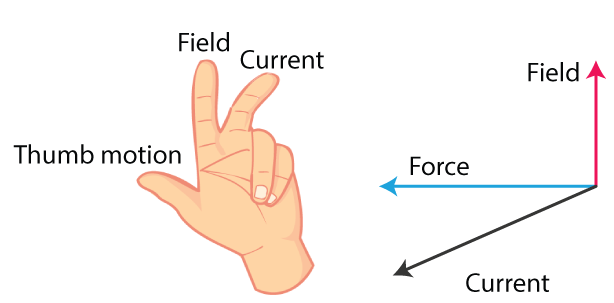Question: 6

State the unit of magnetic field in terms of the force experienced by a current-carrying conductor placed in a magnetic field.

Solution:

The unit of magnetic field in terms of the force experienced by a current-carrying conductor placed in a magnetic field is Newton/ampere × meter (or NA-1m-1)

Question: 7

A flat coil ABCD is freely suspended between the pole pieces of a U-shaped permanent magnet with the plane of coil parallel to the magnetic field.

(a)What happens when a current is passed in the coil?

(b) When will coil come to rest?

(c) When will the couple acting on the coil be (i) maximum, (ii) minimum?

(d) Name an instrument which makes use of the principle stated above.

Solution:

(a) The coil experiences a torque due to which it rotates

(b) The coil will come to rest when its plane becomes normal to the magnetic field

(c) (i) When the plane of the coil is parallel to the magnetic field

(ii) When the plane of the coil is normal to the magnetic field

(d) The instrument which makes use of the principle is d.c. motor

Question: 8

A coil ABCD mounted on an axle is placed between the poles N and S of a permanent magnet as shown in figure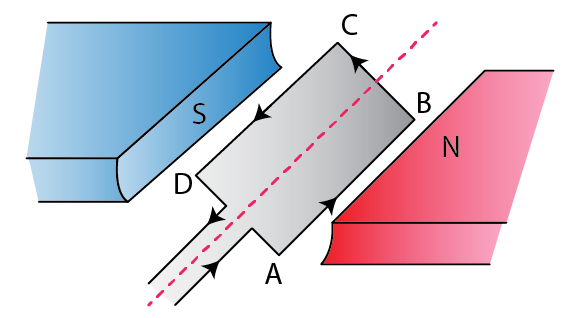(a) In which direction will the coil begin to rotate when current is passed through the coil in direction ABCD by connecting a battery at the ends A and D of the coil?

(b)Why is a commutator necessary for the continuous rotation of the coil?

(c) Complete the diagram with the commutator, etc. for the flow of current in the coil.

Solution:

(a) The coil begins to rotate in an anticlockwise direction

(b) The arms AB and CD get interchanged after half rotation, so the direction of torque on coil reverses. To keep the coil rotating in the same direction, a commutator is needed to reverse the direction of current in the coil after each half rotation of the coil. Hence, the commutator is necessary for the continuous rotation of the coil.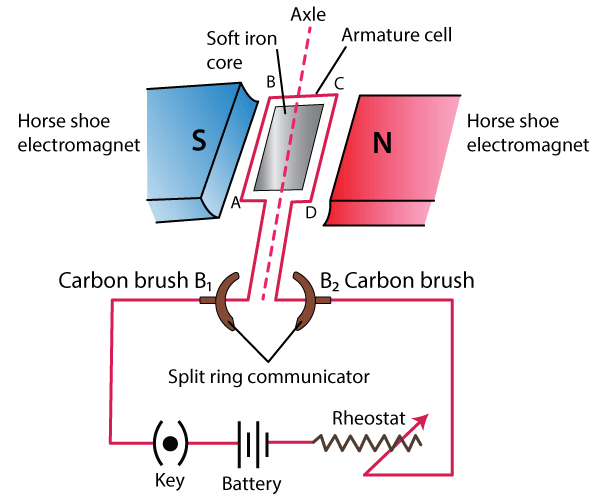Question: 9

What is an electric motor? State its principle.

Solution:

An electric motor is a device which converts the electrical energy into mechanical energy.

Principle: An electric motor (dc motor) works on the principle that when an electric current is passed through a conductor placed normally in a magnetic field, a force acts on the conductor as a result of which the conductor begins to move and thus mechanical energy is obtained.

Question: 10

Draw a labelled diagram of a d.c motor showing its main parts.

Solution: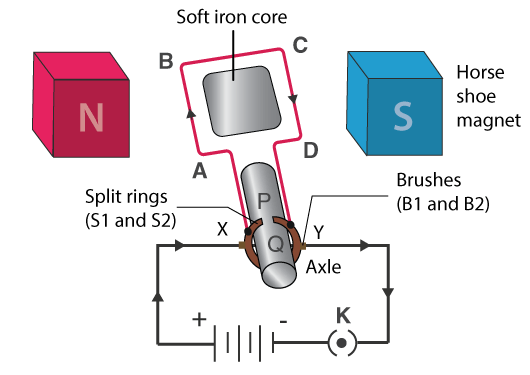Question: 11

What energy conversion does take place during the working of a d.c motor?

Solution:

During the working of a d.c. motor electrical energy converts into mechanical energy.

Question: 12

State two ways by which the speed of rotation of an electric motor can be increased.

Solution:

The speed of rotation of an electric motor can be increased by the following ways

(i) By increasing the strength of the current in the coil

(ii) By increasing the number of turns in the coil

Question: 13

Name two appliances in which an electric motor is used.

Solution:

Electric motors are used in electrical gadgets like fan, washing machines, refrigerators, computers etc.

MULTIPLE CHOICE TYPE

Question: 1

In an electric motor, the energy transformation is:

(a) From electrical to chemical

(b) From chemical to light

(c) From mechanical to electrical

(d) From electrical to mechanical

Solution:

In an electric motor, the energy transformation is from electrical to mechanical.

Exercise 10(c) page no: 258

Question: 1

(a) What is electromagnetic induction?

(b) Describe one experiment to demonstrate the phenomenon of electromagnetic induction.

Solution:

(a) Whenever there is a change in the number of magnetic field lines linked with a conductor, an electromotive force is developed between the ends of the conductor which lasts as long as there is a change in the number of magnetic field lines. This phenomenon is called the electromagnetic induction.

(b) Demonstration of the phenomenon of electromagnetic induction: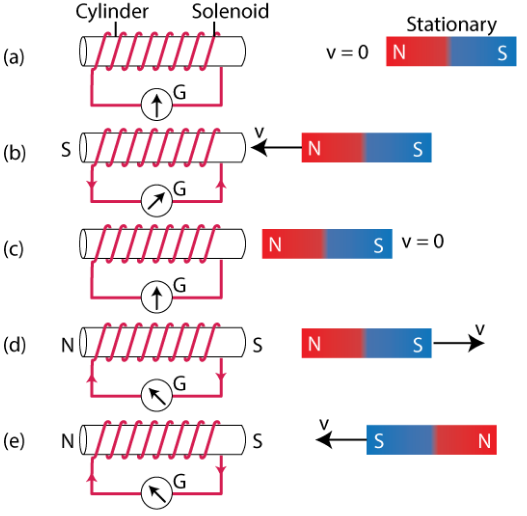(i) When the magnet is stationary, there is no deflection in galvanometer and its pointer reads zero as shown in figure (a)

(ii) When the magnet with its north pole facing the solenoid is moved towards it, the galvanometer shows a deflection towards right showing that a current flows in solenoid in the direction B to A as shown in figure (b)

(iii) The pointer of the galvanometer comes to zero position as the motion of magnet stops. This shows that the current in solenoid flows as long as the magnet remains moving.

(iv) If the magnet is moved away from the solenoid, the pointer of the galvanometer deflects towards left. The current in solenoid flows again, but now in direction A to B which is opposite to that as shown in figure (b)

(v) If the magnet is moved away rapidly, the deflection in the galvanometer increases although the direction of deflection remains the same. It shows that now more current flows.

(vi) If the magnet is brought towards the solenoid by keeping its south pole towards it, the pointer of the galvanometer deflects towards left as shown in figure (e). This shows that the current in solenoid flows in direction A to B which is opposite to that shown in figure (b)

Question: 2

State Faraday’s laws of electromagnetic induction.

Solution:

Faraday formulated the following two laws of electromagnetic induction:

(i) Whenever there is a change in magnetic flux linked with a coil, an e.m.f. is induced. The e.m.f. induced lasts so long there is a change in the magnetic flux linked with the coil.

(ii) The magnitude of e.m.f. induced is directly proportional to the rate of change of magnetic flux linked with the coil. If magnetic flux changes at a constant rate, a steady e.m.f. is produced.

Question: 3

State two factor on which the magnitude of induced e.m.f in a coil depend.

Solution:

The magnitude of induced e.m.f. in a coil depends on the following two factors:

(i) On the rate of change of magnetic flux with each turn, and

(ii) On the number of turns in the coil.

Question: 4

(a)What kind of the energy change takes place when a magnet is moved towards a coil having a galvanometer between its ends?

(b)Name the phenomenon.

Solution:

(a) When a magnet is moved towards a coil having a galvanometer between its ends then mechanical energy changes to the electrical energy.

(b) The phenomenon is known as electromagnetic induction.

Question: 5

(a) How would you demonstrate that a momentary current can be obtained by the suitable use of a magnet, a coil of wire and a galvanometer?

(b)What is the source of energy associated with the current obtained in part (a)?

Solution: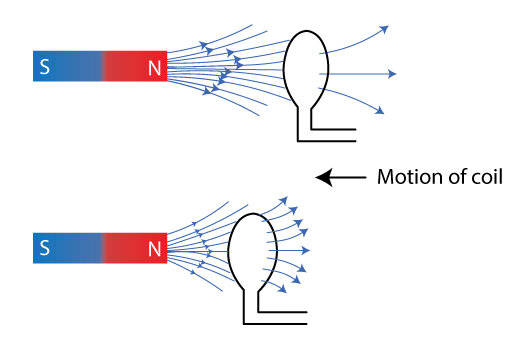(a) When there is a relative motion between the coil and magnet, magnetic flux linked with the coil changes. The magnetic flux through the coil increases if the coil is moved towards the north pole of the magnet. Due to change in the magnetic flux linked with the coil, an e.m.f. is induced in the coil. The induced e.m.f. causes a current to flow in the coil if the circuit of coil is closed.

(b) Mechanical energy is the source of energy associated with the current obtained in part (a)

Question: 6

(a)Describe briefly one way of producing an induced e.m.f.

(b)State one factor that determines the magnitude of induced e.m.f. in part (a) above.

(c)What factor determines the direction of induced e.m.f. in part (a) above?

Solution:

(a) An induced e.m.f. can be produced by the following activity: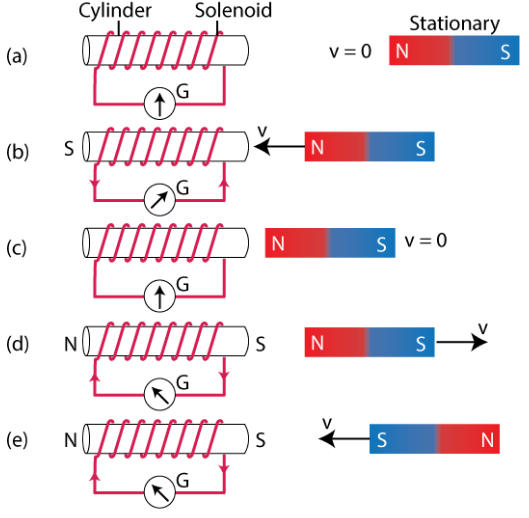(i) When the magnet is stationary, there is no deflection in galvanometer and its pointer reads zero as shown in figure (a)

(ii) When the magnet with its north pole facing the solenoid is moved towards it, the galvanometer shows a deflection towards right showing that a current flows in solenoid in the direction B to A as shown in figure (b)

(iii) The pointer of the galvanometer comes to zero position as the motion of magnet stops. This shows that the current in solenoid flows as long as the magnet remains moving.

(iv) If the magnet is moved away from the solenoid, the pointer of the galvanometer deflects towards left. The current in solenoid flows again, but now in direction A to B which is opposite to that as shown in figure (b)

(v) If the magnet is moved away rapidly, the deflection in the galvanometer increases although the direction of deflection remains the same. It shows that now more current flows.

(vi) If the magnet is brought towards the solenoid by keeping its south pole towards it, the pointer of the galvanometer deflects towards left as shown in figure (e). This shows that the current in solenoid flows in direction A to B which is opposite to that shown in figure (b)

(b) Magnitude of induced e.m.f. depend upon:

(i) On the rate of change of magnetic flux and

(ii) On the number of turns in the coil

(c) The direction of induced e.m.f. determines whether there is an increase or decrease in the magnetic flux.

Question: 7

Complete the following sentences:

The current is induced in a closed circuit only if there is _________

Solution:

The current is induced in a closed circuit only if there is a change in a number of magnetic field lines linked with the circuit.

Question: 8

In which of the following cases e.m.f. is induced?

(i) A current is started in a wire held near a loop of wire.

(ii) The current is switched off in a wire held near a loop of wire.

(iii) A magnet is moved through a loop of wire.

(iv) A loop of wire is held near a magnet.

Solution:

(i) Yes

(ii) Yes

(iii) Yes

(iv) No

Question: 9

A conductor is moved in a varying magnetic field. Name the law which determines the direction of current induced in the conductor.

Solution:

Fleming’s right-hand rule is the law which determines the direction of current induced in the conductor.

Question: 10

State Fleming’s right-hand rule.

Solution:

Fleming’s right-hand rule: Stretch the thumb, central finger and forefinger of your right hand mutually perpendicular to each other. If the forefinger indicates the direction of the magnetic field and the thumb indicates the direction of motion of the conductor, then the central finger will indicate the direction of induced current.

Question: 11

What is Lenz’s law?

Solution:

According to Lenz’s law, the direction of induced e.m.f. (or induced current) is such that it opposes the cause which produces it.

Question: 12

Why does it become more difficult to move a magnet towards a coil when the number of turns in the coil has been increased?

Solution:

The magnitude of induced e.m.f. in the coil becomes more when a coil has a large number of turns and then by Lenz’s law it will oppose more. Hence it becomes more difficult to move a magnet towards a coil.

Question: 13

Explain why an induced current must flow in such a direction so as to oppose the change producing it.

Solution:

An induced current must flow in such a direction so as to oppose the change producing it because mechanical energy is spent in producing the change, which changes into the electrical energy in form of the induced current.

Question: 14

Explain how does the Lenz’s law show the conservation of energy in electromagnetic induction.

Solution:

It shows that the mechanical energy is spent in doing work, against the opposing force experienced by the moving magnet, is transformed into the electrical energy due to which current flows in the solenoid. Thus Lenz’s law is based on the law of conservation of energy.

Question: 15

The diagram shows a coil of several turns of copper wire near a magnet NS. The coil is moved in the direction of the arrow shown in the diagram.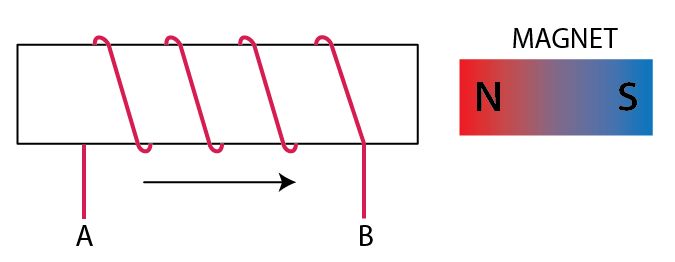(i) In what direction does the induced current flow in the coil?

(ii) Name the law used to arrive at the conclusion in part (i).

(iii) How would the current in coil be altered if

(a) the coil has twice the number of turns,

(b) the coil was made to move three times fast?

Solution:

(i) The North pole generates when the coil is moved in the direction of the magnet and current starts flowing from A to B

(ii) Lenz’s law

(iii) (a) Current is directly proportional to the number of turns. Hence, the current becomes twice.

(b) Current becomes thrice

Question: 16

The diagram shows a fixed coil of several turns connected to a centre zero galvanometer G and a magnet NS which can move in the direction shown in the diagram.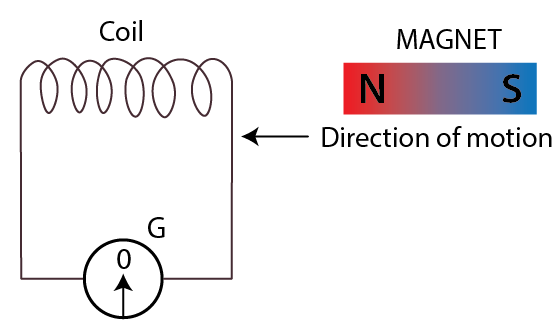(a)Describe the observation in the galvanometer if  (i) the magnet is moved away rapidly, (ii) the magnet is kept stationary after it has moved into the coil, (iii) the magnet is then rapidly pulled out of the coil.

(b) How would the observation in (i) of part (a) change if a more powerful magnet is used?

Solution:

(a) (i) The direction of deflection remains constant in the galvanometer, if the magnet is moved away rapidly

(ii) The deflection becomes zero, if the magnet is kept stationary after it has moved into the coil

(iii) The deflection again occurs in the opposite direction, if the magnet is rapidly pulled out of the coil

(b) The deflection is increased

Question: 17

Name and state the principle of a simple a.c. generator. What is its use?

Solution:

An A.C. generator works on the principle of Faraday’s law of electromagnetic induction

Statement: In a generator, if a coil is rotated in a magnetic field, then due to rotation, the magnetic flux linked with the coil changes and therefore an e.m.f. is induced between the ends of the coil. Thus a generator acts as a source of current in an external circuit containing load when connected between the ends of its coil.

Use: A.C. generator is used to supply power or electricity to a device that requires alternating current.

Question: 18

What determines the frequency of a.c. produced in a generator?

Solution:

The frequency of a.c. produced in a generator determines the number of rotations of the coil in one second or the speed of rotation of the coil.

Question: 19

Complete the sentence:

An a.c. generator changes the ___________ energy to ___________ energy.

Solution:

An a.c. generator changes the mechanical energy to electrical energy.

Question: 20

Draw a labelled diagram of a simple a.c generator.

Solution: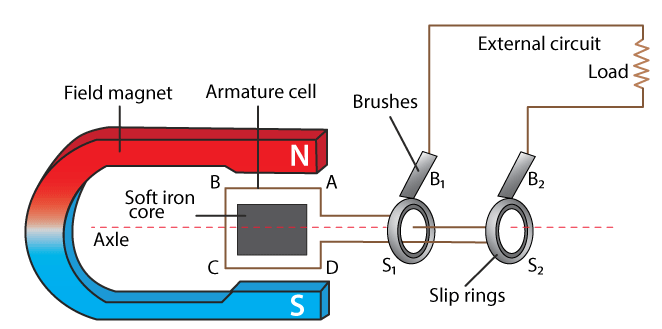Question: 21

In an a.c generator the speed at which the coil rotates is doubled. How would this affect

(a)the frequency of output voltage

(b)the maximum output voltage.

Solution:

(a) The frequency is also doubled in an a.c. generator, if the speed at which the coil rotates is doubled.

(b) The maximum output voltage is also doubled.

Question: 22

State two ways to produce a higher e.m.f. in an a.c generator.

Solution:

Two ways to produce a higher e.m.f. in an a.c. generator are as follows:

(i) By increasing the speed of rotation of the coil

(ii) By increasing the number of turns in the coil

Question: 23

What energy conversion does take place in a generator when it is in use?

Solution:

The mechanical energy changes into the electrical energy in a generator when it is in use.

Question: 24

State (i) two dis-similarities, and (ii) two similarities between a D.C. motor and an A.C. generator.

Solution:

(i) Two dis-similarities between a D.C. motor and an A.C. generator are

 A.C. generator D.C. motor A generator is a device which converts the mechanical energy into the electrical energy A d.c. motor is a device which converts the electrical energy into the mechanical energy A generator works on the principle of electromagnetic induction A d.c. motor works on the principle of force acting on a current-carrying conductor placed in a magnetic field.

(ii) Similarities between an a.c. generator and a d.c. motor are as follows:

(i) Both in an a.c. generator and d.c. motor, a coil rotates in a magnetic field between the pole pieces of a powerful electromagnet.

(ii) Both in an a.c. generator and d.c. motor, there is a transformation of energy from one form to the other form.

Question: 25

State one advantage of using a.c. over d.c.

Solution:

The voltage of a.c. can be increased by the use of step-up transformer at the power generating station and then it can be decreased by the use of a step down transformer for transmission in the city. In the transmission line wires, it reduces the loss of electrical energy as heat. At the power generating station, if d.c. is generated, its voltage cannot be increased for transmission. There will be a huge loss of electrical energy as heat in the line wires, due to passage of high current in the transmission line wires.

Question: 26

For what purpose are the transformers used? Can they be used with a direct current source?

Solution:

Transformers are used to step up or step down the a.c. voltage. No, a transformer cannot be used with a d.c. source.

Question: 27

State two factors on which the magnitude of an induced e.m.f. in the secondary coil of a transformer depends.

Solution:

Factors on which the magnitude of an induced e.m.f. in the secondary coil of a transformer depends are

(i) The ratio of the number of turns in the secondary coil to the number of turns in the primary coil.

(ii) The magnitude of the e.m.f. applied in the primary coil.

Question: 28

How an e.m.f. in the primary and secondary coils of a transformer related with the number of turns in these coils?

Solution:

The relation is

Es / Ep = Ns / Np

where s stands for secondary and p stands for primary

Question: 29

Draw a labelled diagram to show the various components of a step-up transformer.

Solution: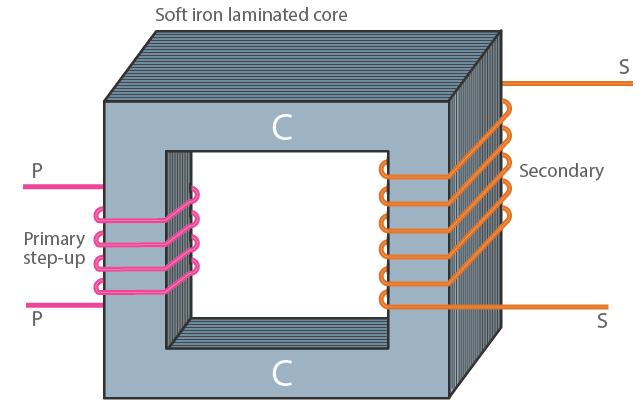Question: 30

Name the device used to transform 12 V a.c. to 200 V a.c. Name the principle on which it works.

Solution:

The device used to transform 12 V a.c. to 200 V a.c. is step-up transformer. It works on the principle of electromagnetic induction.

MULTIPLE CHOICE TYPE

Question: 1

The direction of the induced current is obtained by:

(a) Fleming’s left-hand rule

(b) Clock rule

(c) Right hand thumb rule

(d) Fleming’s right-hand rule

Solution:

The direction of the induced current is obtained by Fleming’s left-hand rule

Question: 2

In a step-up transformer:

(a) Ns = Np

(b) Ns < Np

(c) Ns > Np

(d) nothing can be said

Solution:

In a step-up transformer Ns > Np

NUMERICAL

Question: 1

The magnetic flux through a coil having 100 turns decreases from 5 milli weber to zero in 5 seconds.

Calculate the e.m.f. induced in the coil

Solution:

Given

Decrease in the flux = 0.005 – 0

= 0.005 weber,

Number of turns = 100 turns and

Time = 5 second

e.m.f. induced = N × Rate of increase of magnetic flux

= 100 × 0.005 / 5

= 0.1 V

∴ e.m.f. induced = 0.1 V = 100 mV

Question: 2

The primary coil of a transformer has 800 turns and the secondary coil has 8 turns. It is connected to a 220 V a.c. supply. What will be the output voltage?

Solution:

Given

Number of turns in primary coil Np = 800

Number of turns in secondary coil Ns = 8

Input supply voltage Ep = 220 V

Now,

Ep / Es = Np / Ns

Es = (Ns × Ep) / Np

Es = (8 × 220) / 800

Es = 1760 / 800

Es = 2.2 V

Question: 3

A transformer is designed to give a supply of 8 V to ring a house bell from the 240 V a.c mains. The primary coil has 4800 turns. How many turns will be in the secondary coil?

Solution:

Given

Input ac voltage Ep = 240 V

Number of turns in primary coil Np = 4800

Number of turns in secondary coil Ns =?

Output voltage Es = 8 V

We know that,

Ep / Es = Np / Ns

Ns = (Np × Es) / Ep

Ns = (4800 × 8) / 240

Ns = 38400 / 240

Ns = 160

∴ Number of turns in secondary coil = 160

Question: 4

The input and output voltages of a transformer are 220 V and 44V respectively. Find

(a)the turns ratio

(b)the current in input circuit if the output current is 2 A.

Solution:

(a) We know that,

Ep / Es = Np / Ns

So turns ratio

Ns / Np = 44 / 220

Ns / Np = 1: 5

(b) Output current Is = 2 A

Es = 44 V

Input current Ip =?

Ep = 220 V

We know that,

EsIs = EpIp

Ip = EsIs / Ep

Ip = (44 × 2A) / 220

Ip = 88A / 220

Ip = 0.4 A

Selina Solutions Concise Physics Class 10 Chapter 10 Electromagnetism covers the concept pertaining to the magnetic and electric field. Here the magnetic and electric field can be converted into one another with relative motion. A few points covered in this chapter are Oersted’s experiment on the magnetic effect of electric current, magnetic field and field lines due to current in a straight wire, the rule to find the direction of magnetic field. Numerical problems along with exercise questions are given to assess students knowledge at the end of the chapter

List of subtopics covered in Selina Solutions Concise Physics Class 10 Chapter 10 Electro-magnetism

 Number Subtopic 10.1 Oersted’s experiment on the magnetic effect of electric current 10.2 Magnetic field and field lines due to current in a straight wire 10.3 Rule to find the direction of magnetic field 10.4 Magnetic field due to current in a loop (or circular coil) 10.5 Magnetic field due to a current-carrying cylindrical coil (or solenoid) 10.6 Electromagnet 10.7 Permanent magnet 10.8 Comparison of an Electromagnet with a permanent magnet 10.9 Advantages of an Electro-magnet over a permanent magnet 10.10 Uses of Electromagnet 10.11 Force on a current-carrying conductor in a magnetic field and its application in d.c. motor 10.12 Simple D.C. motor 10.13 Electromagnetic induction 10.14 Faraday’s laws of Electromagnetic induction 10.15 A.C. generator 10.16 Frequency of a.c. in household supplies 10.17 Distinction between an a.c. generator and d.c. motor 10.18 Transformer

List of Exercises

 Name of the exercise Page number 10(A) 239 10(B) 246 10(C) 258

#### Key Features of Selina Solutions Concise Physics Class 10 Chapter 10 Electro-magnetism

• Selina Solutions present students with detailed solutions, solved step by step
• The solutions makes the learning process easier and gripping
• Selina Solutions are the best study tools in explaining complex problems in the simplest way possible
• Selina Solutions are solved by experts and are completely informative and reliable

The given solutions are as per the 2019-20 Concise Selina textbook. The Selina Solutions for the academic year 2020-21 will be updated soon.